• C语言中实现模板函数
千次阅读
2020-07-24 18:06:15

qsort上.qsort的函数原型：
void qsort( void *base, size_t num, size_t width, int (__cdecl *compare )(const void *elem1, const void *elem2 ) );
快排时，只要自己实现相应数据类型的比较函数cmpare就可以了.如果比较int型时,一个典型的compare函数如下：

int cmp(const void *a,const void *b){
return *((int *)a)-*((int *)b);
}

那么,就是说可以利用void *. void *意指未指定类型，也可以理解为任意类型。其他类型的指针可以直接赋值给void *变量，但是void *变量需要强制类型转换为其它指针类型。这个相信大家都知道。那么下面以一个简单的题目为例，来探讨如何在C语言中实现模板函数。
方法1： 利用void *.
在看下面的源程序之前，需要了解几点。首先，在32位平台上，任何类型的指针所占的字节都是4个字节，因为32位机器虚拟内存一般为4G，即2的32次方，只要32位即4个字节就可以足够寻址，sizeof(void *)=4； 其次，虽然各种不同类型的指针所占的空间都为4个字节，但是不同类型的指针所指的空间的字节数却不同(这一点尤为重要，下面的程序我在开始没有调通就因为这点意识不强)。所以，如果你将一个指针强制转换为另一个类型的指针，指针本身所占的字节是不变的，但是，如果对这个指针进行运算，比如 *p,p++,p-=1等一般都是不同的。 再次，函数指针应该了解下，这里不多说。 最后，因为Sandy跟我说，C++开始的时候模板的实现其实就是利用宏替换，在编译的时候确定类型。所以，为了方便，类型也用了预编译指令#define。

#include "stdio.h"
#include "stdlib.h"

//typedef int T;  //或者下面的也可以.
#define  T int

int cmp(const void *a,const void *b){
return *((T *)a)-*((T *)b);
}
/*
//这个FindMin是Sandy写的.felix021也写了个,差不多的就不贴出来的.
void FindMin(const void  *arr,int arr_size,int arrmembersize,int *index,
int (*cmp)(const void *,const void *b)){
int i;
*index=0;
char *p=(char *)arr;
char *tmp=p;
for (i=1;i<arr_size ;i++){
if (cmp(tmp,p)>0){
tmp=p;
}
p+=arrmembersize;
}
(*index)=((int)(tmp-arr))/arrmembersize;
}
*/

int FindMin(const void *arr,int arr_size,int arrmembersize,int (*cmp)(const void *,const void *)){
char *p=(char *)arr;	//可以把指针看作是char *,如果转换为int *,那下面的位移就不正确了.
int index=0;
int i;
for (i=1;i</arr_size><arr_size ;++i){
if (cmp(p+index*arrmembersize,p+i*arrmembersize)>0){
index=i;
}
}

return index;
}

int main(){
int arr[]={2,1,1,2,3,4,5,0,2,3,1,3};
//int *result;
int result;//result保存的是最小值索引.
result=FindMin(arr,12,sizeof(arr),cmp);

printf("%d,%d
",result,arr[result]);
system("PAUSE");
return 0;
}

方法2：利用宏。在编译的时候确定类型。查阅资料的时候，很多都说这种方法比较好，方便调试，也很直观，虽然很啰嗦。

#include <stdio .h>

#ifndef _INT_
#define _INT_
#endif

int cmp(const void *a,const void *b){
#ifdef _INT_
return (*(int *)a-*(int *)b);
#elif _FLOAT_
return (fabs(*(float *)a-*(float *)b)&lt;1e-6)?-1:1;
#elif _DOUBLE_
return (fabs(*(double *a)-*(double *)b)&lt;1e-9)?-1:1;
#endif
}

#ifdef _INT_
void FindMin(int *arr,int arr_size,int *result,int cmp(const void *a,const void *b))
#elif _FLOAT_
void  FindMin(float *arr,int arr_size,float *result,int cmp(const void *a,const void *b))
#elif _DOUBLE_
void  FindMin(double *arr,int arr_size,double *result,int cmp(const void *a,const void *b))
#endif
{
int i;
*result=arr;
for(i=1;i<arr_size ;++i){
if(cmp(&arr[i],result)>0)
*result=arr[i];
}
}

int main(){
int arr1[]={1,2,4,2,1,7};
int result;
FindMin(arr1,6,&result,cmp);
printf("%d
",result);
return 0;
}

方法3：在findmin中，不用强制类型转换为char *，直接利用memcpy内存拷贝过去，这时，还可以在参数列表中保存结果，而不是索引。此方法由CSDN上的ltc_mouse提供。

#include "stdio.h"
#include "stdlib.h"
#include "string.h"

void FindMin(void *arr,int arr_size,int arrmembersize,void * result,
int (*fpCmp)(const void *,const void *b)){
int i;
unsigned char *pSrc = (unsigned char *)arr;
unsigned char *pRes = (unsigned char *)result;
memcpy( (void *)pRes, (const void *)pSrc, arrmembersize );
for (i=1;i</arr_size><arr_size ;i++){
pSrc += arrmembersize;
if ( fpCmp((const void *)pSrc, result)&lt;0 ){
memcpy( (void *)pRes, (const void *)pSrc, arrmembersize );
}
}
}
int cmp_int(const void *a, const void *b)
{
return ( *(int *)a - *(int *)b );
}
int cmp_double(const void *a, const void *b)
{
return ( (fabs(*(double *)a < *(double *)b ))&lt;1e-9) ? -1 : 1; //这个可能要调整下
}
int main()
{
int iArr[]={1,3,5,-1,3,4,7};
double fArr[]={-3.2, 2.3, 7.8, -9.3, 4.7, 10.5};
int iMin;
double fMin;
FindMin( (void *)iArr, sizeof(iArr)/sizeof(iArr), sizeof(iArr), (void *)&iMin, cmp_int );
FindMin( (void *)fArr, sizeof(fArr)/sizeof(fArr), sizeof(fArr), (void *)&fMin, cmp_double);
printf("Min of iArr is %d
", iMin);
printf("Min of fArr is %lf
", fMin);
return 0;
}

更多相关内容
• ## C++函数模板（模板函数）详解

万次阅读 多人点赞 2019-07-04 16:03:01
C++函数模板（模板函数）详解定义用法：函数模板的原理延申用法2.1为什么需要类模板2.2单个类模板语法2.3继承中的类模板语法案例1：案例2：2.4类模板的基础语法2.5类模板语法知识体系梳理1.所有的类模板函数写在类的...

# 定义

函数模板不是一个实在的函数，编译器不能为其生成可执行代码。定义函数模板后只是一个对函数功能框架的描述，当它具体执行时，将根据传递的实际参数决定其功能。（好吧，咱也听不懂，直接上用法吧?）

# 用法：

面向对象的继承和多态机制有效提高了程序的可重用性和可扩充性。在程序的可重用性方面，程序员还希望得到更多支持。举一个最简单的例子，为了交换两个整型变量的值，需要写下面的 Swap 函数：

void Swap(int & x, int & y)
{
int tmp = x;
x = y;
y = tmp;
}


为了交换两个 double 型变量的值，还需要编写下面的 Swap 函数：

void Swap (double & xr double & y)
{
double tmp = x;
x = y;
y = tmp;
}


如果还要交换两个 char 型变量的值，交换两个 CStudent 类对象的值……都需要再编写 Swap 函数。而这些 Swap 函数除了处理的数据类型不同外，形式上都是一样的。能否只写一遍 Swap 函数，就能用来交换各种类型的变量的值呢？继承和多态显然无法解决这个问题。因此，“模板”的概念就应运而生了。

众所周知，有了“模子”后，用“模子”来批量制造陶瓷、塑料、金属制品等就变得容易了。程序设计语言中的模板就是用来批量生成功能和形式都几乎相同的代码的。有了模板，编译器就能在需要的时候，根据模板自动生成程序的代码。从同一个模板自动生成的代码，形式几乎是一样的。

# 函数模板的原理

C++ 语言支持模板。有了模板，可以只写一个 Swap 模板，编译器会根据 Swap 模板自动生成多个 Sawp 函数，用以交换不同类型变量的值。

在 C++ 中，模板分为函数模板和类模板两种。

• 函数模板是用于生成函数；
• 类模板则是用于生成类的。

函数模板的写法如下：

template <class 类型参数1, class类型参数2, ...>
返回值类型  模板名(形参表)
{
函数体
}


其中的 class 关键字也可以用 typename 关键字替换，例如：

template <typename 类型参数1, typename 类型参数2, ...>


函数模板看上去就像一个函数。前面提到的 Swap 模板的写法如下：

template <class T>
void Swap(T & x, T & y)
{
T tmp = x;
x = y;
y = tmp;
}


T 是类型参数，代表类型。编译器由模板自动生成函数时，会用具体的类型名对模板中所有的类型参数进行替换，其他部分则原封不动地保留。同一个类型参数只能替换为同一种类型。编译器在编译到调用函数模板的语句时，会根据实参的类型判断该如何替换模板中的类型参数。

例如下面的程序：

#include <iostream>
using namespace std;
template<class T>
void Swap(T & x, T & y)
{
T tmp = x;
x = y;
y = tmp;
}
int main()
{
int n = 1, m = 2;
Swap(n, m);  //编译器自动生成 void Swap (int &, int &)函数
double f = 1.2, g = 2.3;
Swap(f, g);  //编译器自动生成 void Swap (double &, double &)函数
return 0;
}


编译器在编译到Swap(n, m);时找不到函数 Swap 的定义，但是发现实参 n、m 都是 int 类型的，用 int 类型替换 Swap 模板中的 T 能得到下面的函数：

void Swap (int & x, int & y)
{
int tmp = x;
x = y;
y = tmp;
}


该函数可以匹配Swap(n, m);这条语句。于是编译器就自动用 int 替换 Swap 模板中的 T，生成上面的 Swap 函数，将该 Swap 函数的源代码加入程序中一起编译，并且将Swap(n, m);编译成对自动生成的 Swap 函数的调用。

同理，编译器在编译到Swap(f, g);时会用 double 替换 Swap 模板中的 T，自动生成以下 Swap 函数：

void Swap(double & x, double & y)
{
double tmp = x;
x = y;
y = tmp;
}


然后再将Swap(f, g);编译成对该 Swap 函数的调用。

编译器由模板自动生成函数的过程叫模板的实例化。由模板实例化而得到的函数称为模板函数。在某些编译器中，模板只有在被实例化时，编译器才会检查其语法正确性。如果程序中写了一个模板却没有用到，那么编译器不会报告这个模板中的语法错误。

编译器对模板进行实例化时，并非只能通过模板调用语句的实参来实例化模板中的类型参数，模板调用语句可以明确指明要把类型参数实例化为哪种类型。可以用：

模板名<实际类型参数1, 实际类型参数2, ...>


的方式告诉编译器应该如何实例化模板函数。例如下面的程序：

#include <iostream>
using namespace std;
template <class T>
T Inc(int n)
{
return 1 + n;
}
int main()
{
cout << Inc<double>(4) / 2;
return 0;
}


Inc(4)指明了此处实例化的模板函数原型应为：

double Inc(double);


编译器不会因为实参 4 是 int 类型，就生成原型为 int Inc(int) 的函数。因此，上面程序输出的结果是 2.5 而非 2。

函数模板中可以有不止一个类型参数。例如，下面这个函数模板的写法是合法的：

template <class Tl, class T2>
T2 print(T1 argl, T2 arg2)
{
cout << arg1 << " " << arg2 << endl;
return arg2;
}


【实例】一个求数组中最大元素的函数模板
例题：设计一个分数类 CFraction，再设计一个名为 MaxElement 的函数模板，能够求数组中最大的元素，并用该模板求一个 CFmction 数组中的最大元素。

示例程序如下：

#include <iostream>
using namespace std;
template <class T>
T MaxElement(T a[], int size) //size是数组元素个数
{
T tmpMax = a;
for (int i = 1; i < size; ++i)
if (tmpMax < a[i])
tmpMax = a[i];
return tmpMax;
}
class CFraction //分数类
{
int numerator;   //分子
int denominator; //分母
public:
CFraction(int n, int d) :numerator(n), denominator(d) { };
bool operator <(const CFraction & f) const
{//为避免除法产生的浮点误差，用乘法判断两个分数的大小关系
if (denominator * f.denominator > 0)
return numerator * f.denominator < denominator * f.numerator;
else
return numerator * f.denominator > denominator * f.numerator;
}
bool operator == (const CFraction & f) const
{//为避免除法产生的浮点误差，用乘法判断两个分数是否相等
return numerator * f.denominator == denominator * f.numerator;
}
friend ostream & operator <<(ostream & o, const CFraction & f);
};
ostream & operator <<(ostream & o, const CFraction & f)
{//重载 << 使得分数对象可以通过cout输出
o << f.numerator << "/" << f.denominator; //输出"分子/分母" 形式
return o;
}
int main()
{
int a = { 1,5,2,3,4 };
CFraction f = { CFraction(8,6),CFraction(-8,4),
CFraction(3,2), CFraction(5,6) };
cout << MaxElement(a, 5) << endl;
cout << MaxElement(f, 4) << endl;
return 0;
}


编译到第 41 行时，根据实参 a 的类型，编译器通过 MaxElement 模板自动生成了一个 MaxElement 函数，原型为：

int MaxElement(int a[], int size);


编译到第 42 行时，根据 f 的类型，编译器又生成一个 MaxElement 函数，原型为：

CFraction MaxElement(CFraction a[], int size);


在该函数中，用到了<比较两个 CFraction 对象的大小。如果没有对<进行适当的重载，编译时就会出错。

从 MaxElement 模板的写法可以看出，在函数模板中，类型参数不但可以用来定义参数的类型，还能用于定义局部变量和函数模板的返回值。

# 延申用法

## 2.1为什么需要类模板

• 类模板与函数模板的定义和使用类似，我们已经进行了介绍。 有时，有两个或多个类，其功能是相同的，仅仅是数据类型不同，如下面语句声明了一个类：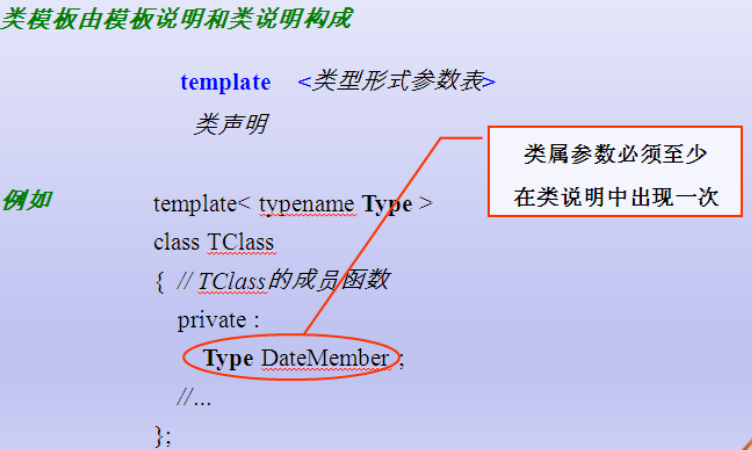• 类模板用于实现类所需数据的类型参数化

• 类模板在表示如数组、表、图等数据结构显得特别重要，

• 这些数据结构的表示和算法不受所包含的元素类型的影响

## 2.2单个类模板语法

 1 //类的类型参数化 抽象的类
2 //单个类模板
3 template<typename T>
4 class A
5 {
6 public:
7     A(T t)
8     {
9         this->t = t;
10     }
11
12     T &getT()
13     {
14         return t;
15     }
16 protected:
17 public:
18     T t;
19 };
20 void main()
21 {
22    //模板了中如果使用了构造函数,则遵守以前的类的构造函数的调用规则
23     A<int>  a(100);
24     a.getT();
25     printAA(a);
26     return ;
27 }


## 2.3继承中的类模板语法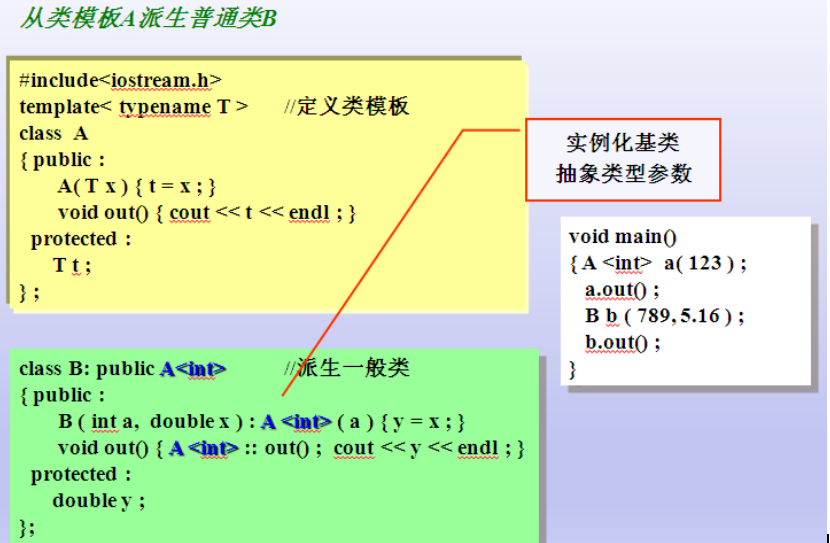### 案例1：

 1 //结论: 子类从模板类继承的时候,需要让编译器知道 父类的数据类型具体是什么(数据类型的本质:固定大小内存块的别名)A<int>
2 //
3 class B : public A<int>
4 {
5 public:
6     B(int i) : A<int>(i)
7     {
8
9     }
10     void printB()
11     {
12         cout<<"A:"<<t<<endl;
13     }
14 protected:
15 private:
16 };
17
18 //模板与上继承
19 //怎么样从基类继承
20 //若基类只有一个带参数的构造函数,子类是如何启动父类的构造函数
21 void pintBB(B &b)
22 {
23     b.printB();
24 }
25 void printAA(A<int> &a)  //类模板做函数参数
26 {
27      //
28     a.getT();
29 }
30
31 void main()
32 {
33     A<int>  a(100); //模板了中如果使用了构造函数,则遵守以前的类的构造函数的调用规则
34     a.getT();
35     printAA(a);
36
37     B b(10);
38     b.printB();
39
40
41     cout<<"hello..."<<endl;
42     system("pause");
43     return ;
44 }


### 案例2：

 1 #include<iostream>
2 using namespace std;
3 //A编程模板类--类型参数化
4 /*
5 类模板的定义 类模板的使用 类模板做函数参数
6 */
7 template <typename T>
8 class A
9 {
10 public:
11     A(T a = 0)
12     {
13         this->a = a;
14     }
15 public:
16     void printA()
17     {
18         cout << "a:" << a << endl;
19     }
20 protected:
21     T a;
22 private:
23
24 };
25 //从模板类派生时，需要具体化模板类，C++编译器需要知道父类的数据类型是什么样子的
26 //要知道父类所占的内存多少
27 class B :public A<int>
28 {
29 public:
30     B(int a =10, int b =20):A<int>(a)
31     {
32         this->b = b;
33     }
34     void printB()
35     {
36         cout << "a:" << a << "b:" << b << endl;
37     }
38 protected:
39 private:
40     int b;
41
42 };
43 //从模板类派生模板类
44 template <typename T>
45 class C :public A<T>
46 {
47
48 public:
49     C(T c,T a) : A<T>(a)
50     {
51         this->c = c;
52     }
53     void printC()
54     {
55         cout << "c:" << c << endl;
56     }
57 protected:
58     T c;
59 private:
60
61 };
62
63 void main()
64 {
65     //B b1(1, 2);
66     //b1.printB();
67     C<int> c1(1,2);
68     c1.printC();
69 }


## 2.4类模板的基础语法

 1 #include<iostream>
2 using namespace std;
3 //A编程模板类--类型参数化
4 /*
5     类模板的定义 类模板的使用 类模板做函数参数
6 */
7 template <typename T>
8 class A
9 {
10 public:
11     A(T a = 0)
12     {
13         this->a = a;
14     }
15 public:
16     void printA()
17     {
18         cout << "a:" << a << endl;
19     }
20 protected:
21 private:
22     T a;
23 };
24 //参数 C++编译器具体的类
25 void UseA(A<int> &a)
26 {
27     a.printA();
28 }
29 void main()
30 {
31     //模板类本身就是抽象的，具体的类，具体的变量
32     A<int> a1(11),a2(22),a3(33);//模板类是抽象的， 需要类型具体化
33     //a1.printA();
34
35     UseA(a1);
36     UseA(a2);
37     UseA(a3);
38 }


## 2.5类模板语法知识体系梳理

### 1.所有的类模板函数写在类的内部

代码：

#### 复数类：

 1 #include<iostream>
2 using namespace std;
3 template <typename T>
4 class Complex
5 {
6 public:
7     friend Complex MySub(Complex &c1, Complex &c2)
8     {
9         Complex tmp(c1.a-c2.a, c1.b-c2.b);
10         return tmp;
11     }
12
13     friend ostream & operator<< (ostream &out, Complex &c3)
14     {
15         out << c3.a << "+" << c3.b <<"i"<< endl;
16         return out;
17     }
18     Complex(T a, T b)
19     {
20         this->a = a;
21         this->b = b;
22     }
23     Complex operator+(Complex &c2)
24     {
25         Complex tmp(a + c2.a, b + c2.b);
26         return tmp;
27     }
28     void printCom()
29     {
30         cout << "a:" << a << " b:" << b << endl;
31     }
32 protected:
33 private:
34     T a;
35     T b;
36 };
37
38 /*
39     重载运算符的正规写法：
40     重载左移<<  右移>> 只能用友元函数，其他的运算符重载都要用成员函数，不要滥用友元函数
41 */
42 //ostream & operator<< (ostream &out, Complex &c3)
43 //{
44 //    out<< "a:" << c3.a << " b:" << c3.b << endl;
45 //    return out;
46 //}
47 void main()
48 {
49     Complex<int>     c1(1,2);
50     Complex<int>     c2(3, 4);
51
52     Complex<int> c3 = c1 + c2;//重载加号运算符
53
54     c3.printCom();
55
56     //重载左移运算符
57     cout << c3 << endl;
58
59     {
60         Complex<int> c4 = MySub(c1 , c2);
61
62         cout << c4 << endl;
63     }
64     system("pause");
65 }


### 2.所有的类模板函数写在类的外部，在一个cpp中

注意：

复制代码
//构造函数 没有问题

• 普通函数 没有问题

• 友元函数：用友元函数重载 << >>

friend ostream& operator<< (ostream &out, Complex &c3) ;

• 友元函数：友元函数不是实现函数重载（非 << >>）

1 需要在类前增加 类的前置声明 函数的前置声明

template<typename T>

class Complex;

template<typename T>

Complex<T> mySub(Complex<T> &c1, Complex<T> &c2);


2 类的内部声明 必须写成:

friend Complex<T> mySub <T> (Complex<T> &c1, Complex<T> &c2);


3 友元函数实现 必须写成：

template<typename T>

Complex<T> mySub(Complex<T> &c1, Complex<T> &c2)

{

Complex<T> tmp(c1.a - c2.a, c1.b-c2.b);

return tmp;

}


4 友元函数调用 必须写成

Complex<int> c4 = mySub<int>(c1, c2)；

cout<<c4;


结论：友元函数只用来进行 左移 友移操作符重载。

复数类：

代码：

 1 #include<iostream>
2 using namespace std;
3
4 template<typename T>
5 class Complex;
6 template<typename T>
7 Complex<T> mySub(Complex<T> &c1, Complex<T> &c2);
8
9 template <typename T>
10 class Complex
11 {
12 public:
13     friend Complex<T> mySub <T>(Complex<T> &c1, Complex<T> &c2);
14
15     friend ostream & operator<< <T>(ostream &out, Complex &c3);
16     Complex(T a, T b);
17     void printCom();
18     Complex operator+(Complex &c2);
19     Complex operator-(Complex &c2);
20
21 protected:
22 private:
23     T a;
24     T b;
25 };
26
27 //构造函数的实现，写在了外部
28 template <typename T>
29 Complex<T>::Complex(T a, T b)
30 {
31     this->a = a;
32     this->b = b;
33 }
34
35 template <typename T>
36 void Complex<T>::printCom()
37 {
38     cout << "a:" << a << " b:" << b << endl;
39 }
40 //成员函数实现加号运算符重载
41 template <typename T>
42 Complex<T> Complex<T>::operator+(Complex<T> &c2)
43 {
44     Complex tmp(a + c2.a, b + c2.b);
45     return tmp;
46 }
47 template <typename T>
48 Complex<T> Complex<T>::operator-(Complex<T> &c2)
49 {
50     Complex(a-c2.a,a-c2.b);
51     return tmp;
52 }
53 //友元函数实现<<左移运算符重载
54
55 /*
56 严重性    代码    说明    项目    文件    行    禁止显示状态
57 错误    C2768    “operator <<”: 非法使用显式模板参数    泛型编程课堂操练
58
59 错误的本质：两次编译的函数头，第一次编译的函数头，和第二次编译的函数有不一样
60 */
61 template <typename T>
62 ostream & operator<< (ostream &out, Complex<T> &c3)//不加T
63 {
64     out << c3.a << "+" << c3.b << "i" << endl;
65     return out;
66 }
67
68
69 //
70 template <typename T>
71 Complex<T> mySub(Complex<T> &c1, Complex<T> &c2)
72 {
73     Complex<T> tmp(c1.a - c2.a, c1.b - c2.b);
74     return tmp;
75 }
76
77 void main()
78 {
79     Complex<int>     c1(1, 2);
80     Complex<int>     c2(3, 4);
81
82     Complex<int> c3 = c1 + c2;//重载加号运算符
83
84     c3.printCom();
85
86     //重载左移运算符
87     cout << c3 << endl;
88
89     {
90         Complex<int> c4 = mySub<int>(c1, c2);
91
92         cout << c4 << endl;
93     }
94     system("pause");
95 }


所有的类模板函数写在类的外部，在不同的.h和.cpp中
也就是类模板函数说明和类模板实现分开

//类模板函数

构造函数

普通成员函数

友元函数

用友元函数重载<<>>;

用友元函数重载非<< >>

demo_complex.cpp

 1 #include"demo_09complex.h"
2 #include<iostream>
3 using namespace std;
4
5 template <typename T>
6 Complex<T>::Complex(T a, T b)
7 {
8     this->a = a;
9     this->b = b;
10 }
11
12 template <typename T>
13 void Complex<T>::printCom()
14 {
15     cout << "a:" << a << " b:" << b << endl;
16 }
17 //成员函数实现加号运算符重载
18 template <typename T>
19 Complex<T> Complex<T>::operator+(Complex<T> &c2)
20 {
21     Complex tmp(a + c2.a, b + c2.b);
22     return tmp;
23 }
24 //template <typename T>
25 //Complex<T> Complex<T>::operator-(Complex<T> &c2)
26 //{
27 //    Complex(a - c2.a, a - c2.b);
28 //    return tmp;
29 //}
30 template <typename T>
31 ostream & operator<< (ostream &out, Complex<T> &c3)//不加T
32 {
33     out << c3.a << "+" << c3.b << "i" << endl;
34     return out;
35 }
36
37
38 //
39 //template <typename T>
40 //Complex<T> mySub(Complex<T> &c1, Complex<T> &c2)
41 //{
42 //    Complex<T> tmp(c1.a - c2.a, c1.b - c2.b);
43 //    return tmp;
44 //}


demo_09complex.h

 1 #pragma once
2 #include<iostream>
3 using namespace std;
4 template <typename T>
5 class Complex
6 {
7 public:
8     //friend Complex<T> mySub <T>(Complex<T> &c1, Complex<T> &c2);
9
10     friend ostream & operator<< <T>(ostream &out, Complex &c3);
11     Complex(T a, T b);
12     void printCom();
13     Complex operator+(Complex &c2);
14     //Complex operator-(Complex &c2);
15
16 protected:
17 private:
18     T a;
19     T b;
20 };


demo_09complex_text.cpp

 1 #include"demo_09complex.h"
2 #include"demo_09complex.cpp"
3
4 #include<iostream>
5 using namespace std;
6
7 void main()
8 {
9     Complex<int>     c1(1, 2);
10     Complex<int>     c2(3, 4);
11
12     Complex<int> c3 = c1 + c2;//重载加号运算符
13
14     c3.printCom();
15
16     //重载左移运算符
17     cout << c3 << endl;
18
19     /*{
20         Complex<int> c4 = mySub<int>(c1, c2);
21
22         cout << c4 << endl;
23     }*/
24     system("pause");
25 }


## 2.5总结

归纳以上的介绍，可以这样声明和使用类模板：

1. 先写出一个实际的类。由于其语义明确，含义清楚，一般不会出错。
2. 将此类中准备改变的类型名(如int要改变为float或char)改用一个自己指定的虚拟类型名(如上例中的numtype)。
3. 在类声明前面加入一行，格式为：

  template <class 虚拟类型参数>

如：

template <class numtype> //注意本行末尾无分号

class Compare

{…}; //类体

1. 用类模板定义对象时用以下形式：

 类模板名<实际类型名> 对象名;

类模板名<实际类型名> 对象名(实参表列);

如：

Compare<int> cmp;

Compare<int> cmp(3,7);

1. 如果在类模板外定义成员函数，应写成类模板形式：

 template <class 虚拟类型参数>

函数类型 类模板名<虚拟类型参数>::成员函数名(函数形参表列) {…}


### 关于类模板的几点说明：

1. 类模板的类型参数可以有一个或多个，每个类型前面都必须加class，如：

template <class T1,class T2>

class someclass

{…};


在定义对象时分别代入实际的类型名，如：

someclass<int,double> obj;

2. 和使用类一样，使用类模板时要注意其作用域，只能在其有效作用域内用它定义对象。

3. 模板可以有层次，一个类模板可以作为基类，派生出派生模板类。

## 2.6类模板中的static关键字

从类模板实例化的每个模板类有自己的类模板数据成员，该模板类的所有对象共享一个static数据成员和非模板类的static数据成员一样，模板类的static数据成员也应该在文件范围定义和初始化
每个模板类有自己的类模板的static数据成员副本

 1 #include<iostream>
2 using namespace std;
3 template <typename T>
4 class AA
5 {
6 public:
7     static T m_a;
8 protected:
9 private:
10 };
11 template <typename T>
12 T AA<T>::m_a =0;
13 void main()
14 {
15     AA<int> a1, a2, a3;
16     a1.m_a = 10;
17     a2.m_a++;
18     a3.m_a++;
19     cout << AA<int>::m_a << endl;
20
21     AA<char> b1, b2, b3;
22     b1.m_a = 'a';
23     b2.m_a++;
24     b3.m_a++;
25     cout << AA<char>::m_a << endl;
26
27     //m_a是每个类型的类，去使用，手工写两个类 int  char
28     system("pause");
29 }


### 案例2：以下来自：C++类模板遇上static关键字

 1 #include <iostream>
2 using namespace std;
3
4 template<typename T>
5 class Obj{
6 public:
7     static T m_t;
8 };
9
10 template<typename T>
11 T Obj<T>::m_t = 0;
12
13 int main04(){
14     Obj<int> i1,i2,i3;
15     i1.m_t = 10;
16     i2.m_t++;
17     i3.m_t++;
18     cout << Obj<int>::m_t<<endl;
19
20     Obj<float> f1,f2,f3;
21     f1.m_t = 10;
22     f2.m_t++;
23     f3.m_t++;
24     cout << Obj<float>::m_t<<endl;
25
26     Obj<char> c1,c2,c3;
27     c1.m_t = 'a';
28     c2.m_t++;
29     c3.m_t++;
30     cout << Obj<char>::m_t<<endl;
31 }


当类模板中出现static修饰的静态类成员的时候，我们只要按照正常理解就可以了。static的作用是将类的成员修饰成静态的，所谓的静态类成员就是指类的成员为类级别的，不需要实例化对象就可以使用，而且类的所有对象都共享同一个静态类成员，因为类静态成员是属于类而不是对象。那么，类模板的实现机制是通过二次编译原理实现的。c++编译器并不是在第一个编译类模板的时候就把所有可能出现的类型都分别编译出对应的类（太多组合了），而是在第一个编译的时候编译一部分，遇到泛型不会替换成具体的类型（这个时候编译器还不知道具体的类型），而是在第二次编译的时候再将泛型替换成具体的类型（这个时候编译器知道了具体的类型了）。由于类模板的二次编译原理再加上static关键字修饰的成员，当它们在一起的时候实际上一个类模板会被编译成多个具体类型的类，所以，不同类型的类模板对应的static成员也是不同的（不同的类），但相同类型的类模板的static成员是共享的（同一个类）。

## 2.7类模板在项目开发中的应用

小结

• 模板是C++类型参数化的多态工具。C++提供函数模板和类模板。

• 模板定义以模板说明开始。类属参数必须在模板定义中至少出现一次。

• 同一个类属参数可以用于多个模板。

• 类属参数可用于函数的参数类型、返回类型和声明函数中的变量。

• 模板由编译器根据实际数据类型实例化，生成可执行代码。实例化的函数。

• 模板称为模板函数；实例化的类模板称为模板类。

• 函数模板可以用多种方式重载。

• 类模板可以在类层次中使用 。

## 训练题

### 1） 请设计一个数组模板类（ MyVector ），完成对int、char、Teacher类型元素的管理。

设计:

• 类模板 构造函数 拷贝构造函数 << [] 重载=操作符

• a2=a1

• 实现

### 2） 请仔细思考：

a) 如果数组模板类中的元素是Teacher元素时，需要Teacher类做什么工作

b) 如果数组模板类中的元素是Teacher元素时，Teacher类含有指针属性哪？

 1 class Teacher
2 {
3     friend ostream & operator<<(ostream &out, const Teacher &obj);
4 public:
5     Teacher(char *name, int age)
6     {
7         this->age = age;
8         strcpy(this->name, name);
9     }
10
11     Teacher()
12     {
13         this->age = 0;
14         strcpy(this->name, "");
15     }
16
17 private:
18     int age;
19     char name;
20 };
21
22
23 class Teacher
24 {
25     friend ostream & operator<<(ostream &out, const Teacher &obj);
26 public:
27     Teacher(char *name, int age)
28     {
29         this->age = age;
30         strcpy(this->name, name);
31     }
32
33     Teacher()
34     {
35         this->age = 0;
36         strcpy(this->name, "");
37     }
38
39 private:
40     int age;
41     char *pname;
42 };


结论1： 如果把Teacher放入到MyVector数组中，并且Teacher类的属性含有指针，就是出现深拷贝和浅拷贝的问题。

结论2：需要Teacher封装的函数有：

1. 重写拷贝构造函数

2. 重载等号操作符

3. 重载左移操作符。

理论提高：

所有容器提供的都是值（value）语意，而非引用（reference）语意。容器执行插入元素的操作时，内部实施拷贝动作。所以STL容器内存储的元素必须能够被拷贝（必须提供拷贝构造函数）。

### 3） 请从数组模板中进行派生

 1 //演示从模板类 派生 一般类
2 #include "MyVector.cpp"
3
4 class MyArray01 : public MyVector<double>
5 {
6 public:
7     MyArray01(int len) : MyVector<double>(len)
8     {
9         ;
10     }
11 protected:
12 private:
13 };
14
15
16 //演示从模板类 派生 模板类 //BoundArray
17 template <typename T>
18 class MyArray02 : public MyVector<T>
19 {
20 public:
21     MyArray02(int len) : MyVector<double>(len)
22     {
23         ;
24     }
25 protected:
26 private:
27 };
28 测试案例：
29
30 //演示 从模板类 继承 模板类
31 void main()
32 {
33     MyArray02<double> dArray2(10);
34     dArray2 = 3.15;
35
36 }
37
38
39 //演示 从模板类 继承 一般类
40 void main11()
41 {
42     MyArray01 d_array(10);
43
44     for (int i=0; i<d_array.getLen(); i++)
45     {
46         d_array[i] = 3.15;
47     }
48
49     for (int i=0; i<d_array.getLen(); i++)
50     {
51         cout << d_array[i] << " ";
52     }
53
54     cout<<"hello..."<<endl;
55     system("pause");
56     return ;
57 }


## 作业：

封装你自己的数组类；设计被存储的元素为类对象；

思考：类对象的类，应该实现的功能。

1. 优化Teacher类, 属性变成 char *panme, 构造函数里面 分配内存

2. 优化Teacher类,析构函数 释放panme指向的内存空间

3. 优化Teacher类,避免浅拷贝 重载= 重写拷贝构造函数

4. 优化Teacher类,在Teacher增加 <<

5. 在模板数组类中,存int char Teacher Teacher*(指针类型)

### zuoye.h

 1 #pragma once
2
3 template <typename T>
4 class MyVector
5 {
6
7     //friend ostream & operator<< <T>(ostream &out, const MyVector &obj);
8 public:
9     MyVector(int size = 0);//构造函数
10     MyVector(const MyVector &obj);//copy构造函数
11     ~MyVector();
12 public:
13     T& operator [](int index);
14     MyVector &operator=(const MyVector &obj);
15
16
17     int getLen()
18     {
19         return m_len;
20     }
21 protected:
22 private:
23     T *m_space;
24     int m_len;
25
26 };


### zuoye_test12.cpp

  1 #include"zuoye.h"
2 #include"zuoye12.cpp"
3 #include<iostream>
4 using namespace std;
5 class Teacher
6 {
7 public:
8     Teacher()
9     {
10         age = 33;
11         m_p = new char;
12         strcpy(m_p, " ");
13     }
14
15     Teacher(char *name, int age)
16     {
17         this->age = age;
18         m_p = new char[strlen(name)+1];
19         strcpy(this->m_p, name);
20
21     }
22     Teacher(const Teacher &obj)
23     {
24         m_p = new char[strlen(obj.m_p) + 1];
25         strcpy(this->m_p, obj.m_p);
26         age = obj.age;
27     }
28     ~Teacher()
29     {
30         if (m_p!=NULL)
31         {
32             delete[] m_p;
33             m_p = NULL;
34         }
35     }
36     void printT()
37     {
38         cout << m_p << ", " << age;
39     }
40 public:
41     //重载<< ==
42     friend ostream & operator<<(ostream &out,Teacher &t);
43     Teacher & operator=(const Teacher &obj)
44     {
45         //1
46         if (m_p!=NULL)
47         {
48             delete[] m_p;
49             m_p = NULL;
50             age = 33;
51         }
52         //2
53         m_p = new char[strlen(obj.m_p) + 1];
54         age = obj.age;
55         //3
56         strcpy(this->m_p, obj.m_p);
57         return *this;
58     }
59 protected:
60 private:
61     int age;
62     //char name;
63     char *m_p;
64 };
65 ostream & operator<<(ostream &out, Teacher &t)
66 {
67     out << t.m_p << ", " << t.age << endl;
68     return out;
69 }
70
71 void main()
72 {
73     Teacher t1("t1", 31), t2("t2", 32);
74
75     MyVector<Teacher *> Tarray(2);
76
77     Tarray = &t1;
78     Tarray = &t2;
79     for (int i = 0; i < 2; i++)
80     {
81         Teacher *tmp = Tarray[i];
82         tmp->printT();
83     }
84     system("pause");
85 }
86 void main123()
87 {
88     Teacher t1("t1", 31), t2("t2", 32);
89     MyVector<Teacher> Tarray(2);
90     Tarray = t1;
91     Tarray = t2;
92     for (int i = 0; i < 2; i++)
93     {
94         Teacher tmp = Tarray[i];
95         tmp.printT();
96     }
97     system("pause");
98 }
99 void main112()
100 {
101     MyVector<int>  myv1(10);
102     myv1 = 'a';
103     myv1 = 'b';
104     myv1 = 'c';
105     myv1 = 'd';
106     myv1 = 'e';
107     //cout << myv1;
108     MyVector<int>  myv2 = myv1;
109 }
110
111
112 void main111()
113 {
114     MyVector<int>  myv1(10);
115     for (int i = 0; i < myv1.getLen(); i++)
116     {
117         myv1[i] = i + 1;
118         cout << myv1[i] << " ";
119     }
120
121     MyVector<int>  myv2 = myv1;
122     for (int i = 0; i < myv2.getLen(); i++)
123     {
124         myv2[i] = i + 1;
125         cout << myv2[i] << " ";
126     }
127
128     //cout << myv2 << endl;//重载左移运算符
129
130     system("pause");
131 }


### zuoye12.cpp

 1 #include"zuoye.h"
2 #include<iostream>
3 using namespace std;
4
5 template <typename T>
6 ostream & operator<<(ostream &out, const MyVector<T> &obj)
7 {
8     for (int i = 0; i<obj.m_len; i++)
9     {
10         out << obj.m_space[i] << " ";
11     }
12     out << endl;
13     return out;
14 }
15 //构造函数
16 template <typename T>
17 MyVector<T>::MyVector(int size = 0)
18 {
19     m_space = new T[size];
20     m_len = size;
21 }
22 //MyVector<int>  myv2 = myv1;
23 template <typename T>
24 MyVector<T>::MyVector(const MyVector &obj)
25 {
26     //根据大小分配内存
27     m_len = obj.m_len;
28     m_space = new T[m_len];
29     //copy数据
30     for (int i = 0; i<m_len; i++)
31     {
32         m_space[i] = obj.m_space[i];
33     }
34 }
35 template <typename T>
36 MyVector<T>::~MyVector()
37 {
38     if (m_space != NULL)
39     {
40         delete[] m_space;
41         m_space = NULL;
42         m_len = 0;
43
44     }
45 }
46 template <typename T>
47 T& MyVector<T>::operator [](int index)
48 {
49     return m_space[index];
50 }
51 template <typename T>
52 MyVector<T> & MyVector<T>::operator=(const MyVector<T> &obj)
53 {
54     //先把a2的内存释放掉
55     if (m_space != NULL)
56     {
57         delete[] m_space;
58         m_space = NULL;
59         m_len = 0;
60
61     }
62
63     //根据a1分配内存
64     m_len = obj.m_len;
65     m_space = new T[m_len];
66
67     //copy数据
68     for (i = 0; i<m_len; i += )
69     {
70         m_space[i] = obj.m_space[i];
71     }
72     return *this;//a2= a1 返回a2的自身
73 }


参考一些资料，加上一些见解，如果有雷同，纯属巧合。
更多C++相关知识体系，请移步C++知识目录

展开全文• 函数模板调用模板函数函数模板和函数重载 前言 C++提供了模板(template)编程的概念。所谓模板，实际上是建立一个通用函数或类，其类内部的类型和函数的形参类型不具体指定，用一个虚拟的类型来代表。这种通用的方式...

# 前言

C++提供了模板(template)编程的概念。所谓模板，实际上是建立一个通用函数或类，其类内部的类型和函数的形参类型不具体指定，用一个虚拟的类型来代表。这种通用的方式称为模板。模板是泛型编程的基础,泛型编程即以一种独立于任何特定类型的方式编写代码。

# C++函数模板的使用

为什么要有函数模板

例如，在一个项目中，有个项目需求是能够实现多个函数用来返回两个数的最大值，要求能支持char类型、int类型、double类型变量。然后呢，根据这个需求，我们写了以下这个代码。

#include <iostream>

using namespace std;

//比较int 类型
int Max(int a, int b)
{
return a > b ? a : b;
}

//比较char 类型
char Max(char a, char b)
{
return a > b ? a : b;
}

//比较float 类型
float Max(float a, float b)
{
return a > b ? a : b;
}

int main(void)
{

int  n = 1;
int	 m = 2;
cout << "max(1, 2) = " << Max(n, m) << endl;

float a = 2.0;
float b = 3.0;
cout << "max(2.0, 3.0) = " << Max(a, b) << endl;

char i = 'a';
char j = 'b';
cout << "max('a', 'b') = " << Max(i, j) << endl;

return 0;
}


执行得到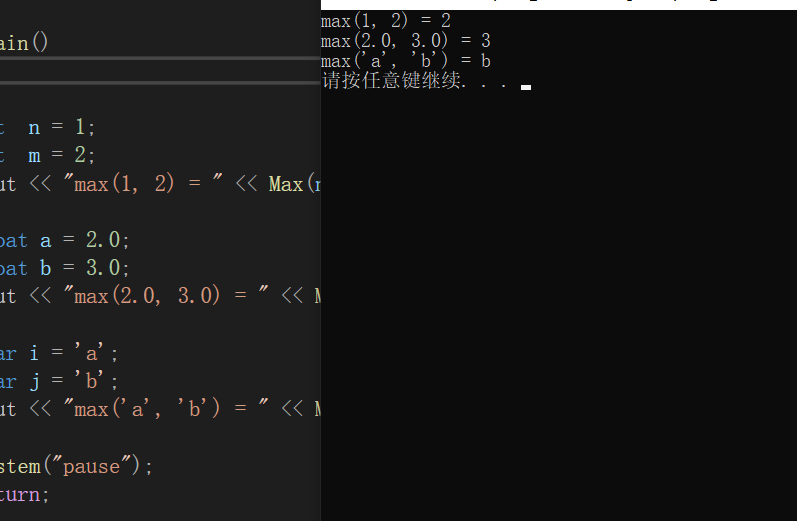我们看到，如果我们需要比较多个类型时，需要多个函数，而实际上我们只需要定义一个 “函数” 就能解决。

#include <iostream>

using namespace std;

//template 关键字告诉C++编译器 要开始泛型编程了
//T - 参数化数据类型
template <typename T>
T Max(T a, T b) {
return a > b ? a : b;
}

int main(void)
{

int  n = 1;
int	 m = 2;
cout << "max(1, 2) = " << Max(n, m) << endl;

float a = 2.0;
float b = 3.0;
cout << "max(2.0, 3.0) = " << Max(a, b) << endl;

char i = 'a';
char j = 'b';
cout << "max('a', 'b') = " << Max(i, j) << endl;

return 0;
}


得到的结果是一样的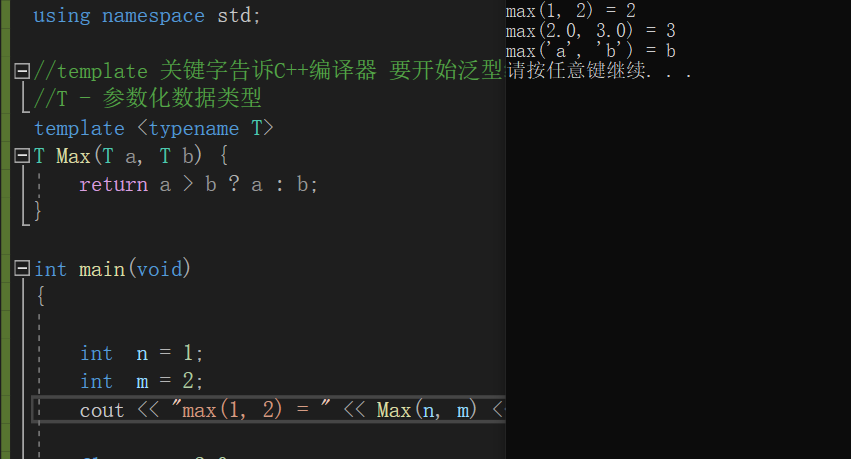我们在定义一个函数模板之后，，没有指定类型，编译器会实现参数类型的自动推导。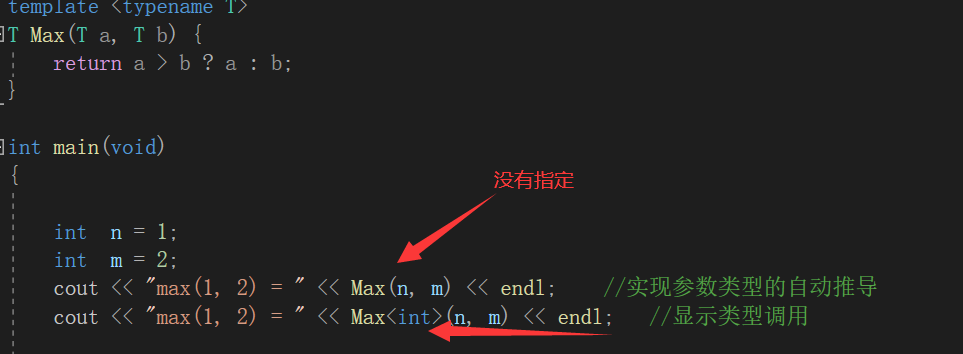# 函数模板语法

所谓函数模板，实际上是建立一个通用函数，其函数类型和形参类型不具体指定，用一个虚拟的类型来代表。这个通用函数就称为函数模板。

凡是函数体相同的函数都可以用这个模板来代替，不必定义多个函数，只需在模板中定义一次即可。在调用函数时系统会根据实参的类型来取代模板中的虚拟类型，从而实现了不同函数的功能。

函数模板定义形式
由以下三部分组成： 模板说明 + 函数定义 + 函数模板调用

template < 类型形式参数表 >
类型 函数名 (形式参数表)
{
//语句序列
}

我们也可以定义多个类型

template <typename T, typename T2>


但是我们定义了一定要用，否则会报错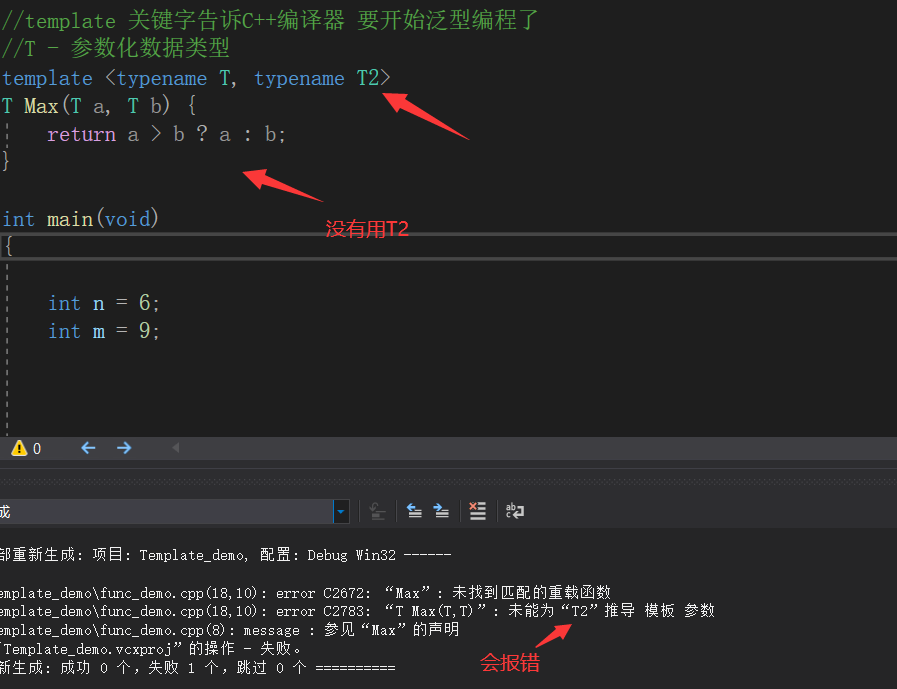将代码改成

#include <iostream>

using namespace std;

//template 关键字告诉C++编译器 要开始泛型编程了
//T - 参数化数据类型
template <typename T, typename T2>
T Max(T a, T2 b) {
return a > b ? a : b;
}

int main(void){

int n = 6;
int m = 9;

cout << Max(n, m) << endl;

system("pause");
return 0;
}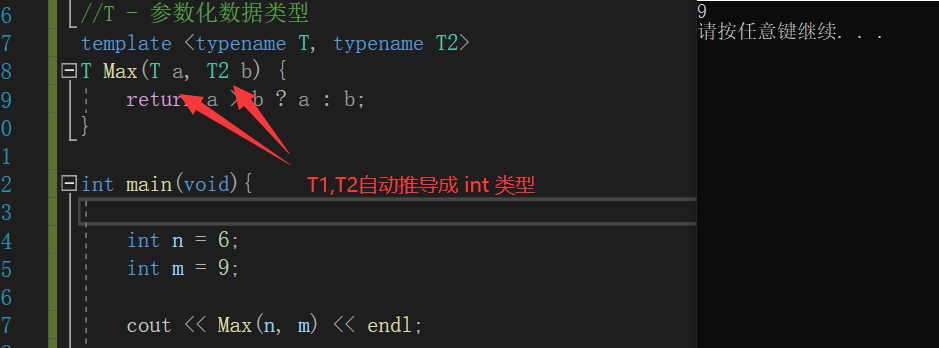## 1.模板说明

template < 类型形式参数表 >
类型形式参数的形式：
typename T1 , typename T2 , …… , typename Tn
或 class T1 , class T2 , …… , class Tn
注意： typenameclass 的效果是一样的，不过建议呢使用typename，为了防止用class有歧义）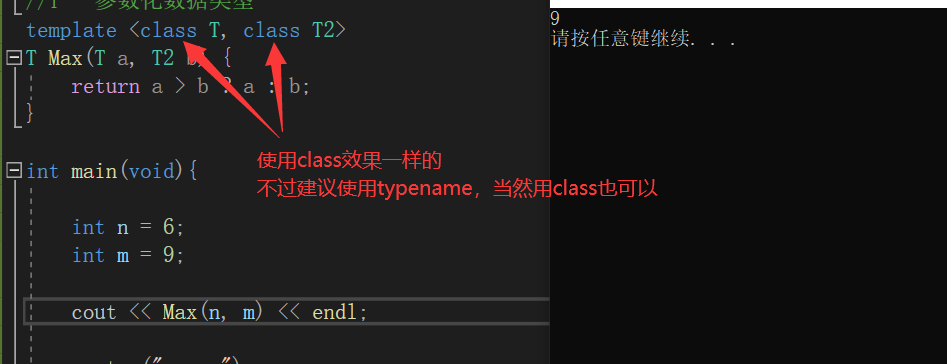## 2.函数定义

类型 函数名 (形式参数表)
{
}
注意： 模板说明的类属参数必须在函数定义中出现一次,函数参数表中可以使用类属类型参数，也可以使用一般类型参数

## 3.函数模板调用

Max<int,int>(a, b); //显式类型调用
Max(a, b); //自动数据类型推导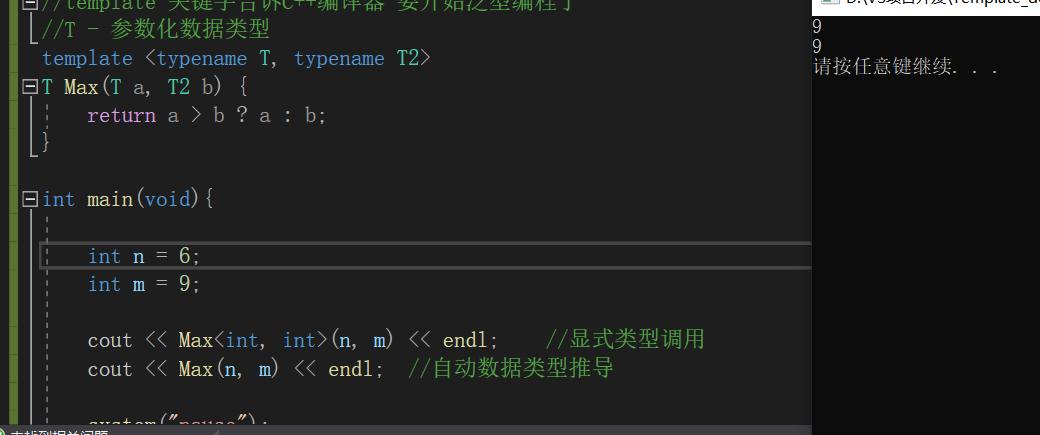我们再来看以下代码

#include <iostream>

using namespace std;

//template 关键字告诉C++编译器 要开始泛型编程了
//T - 参数化数据类型
template <typename T, typename T2>
T Max(T a, T2 b) {
return a > b ? a : b;
}

int main(void){

int n = 6;
int m = 9;
char a = 'c';	//'c' 对应的ascll码值是 99

cout << "Max<int, char>(m, a): " << Max<int, char>(m, a) << endl;	//显式类型调用
cout << "Max(m, a): " << Max(m, a) << endl;	//自动数据类型推导

cout << "Max(a, m): " << Max(a, m) << endl;	//自动数据类型推导

return 0;
}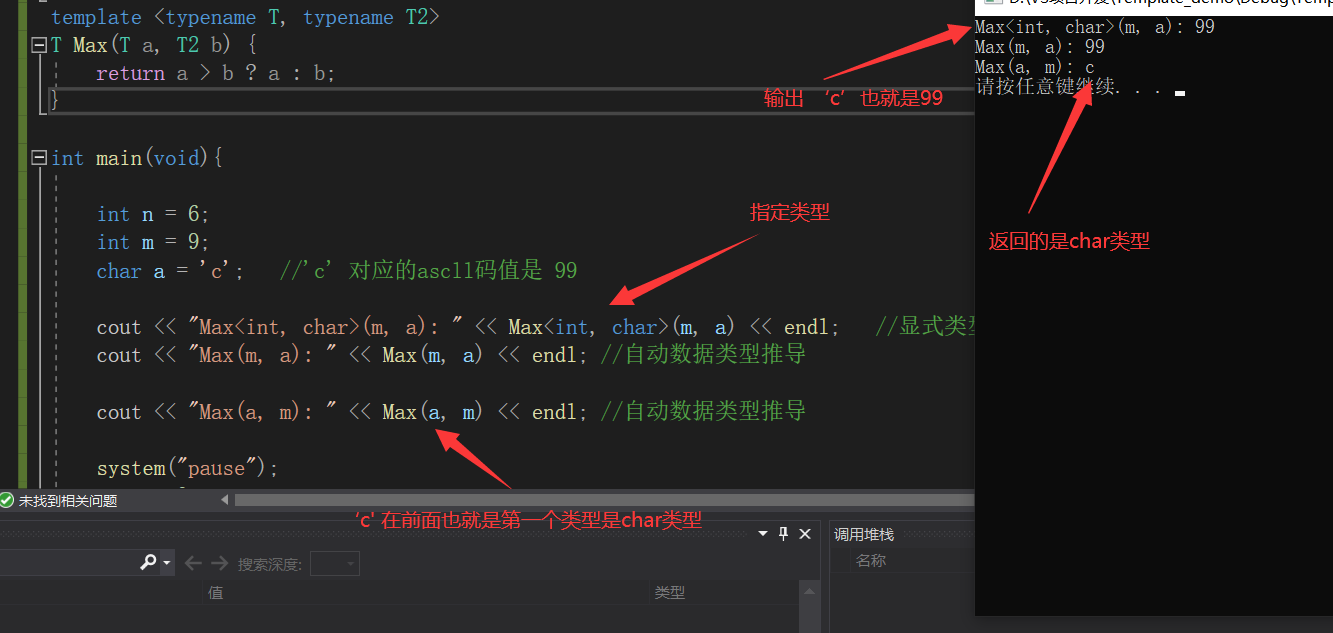为什么会这样呢？因为我们在定义函数模板的时候第一个类型作为了函数类型。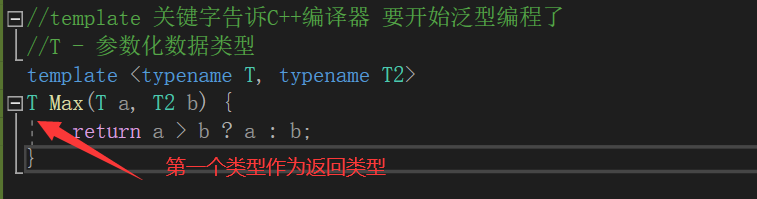# 模板函数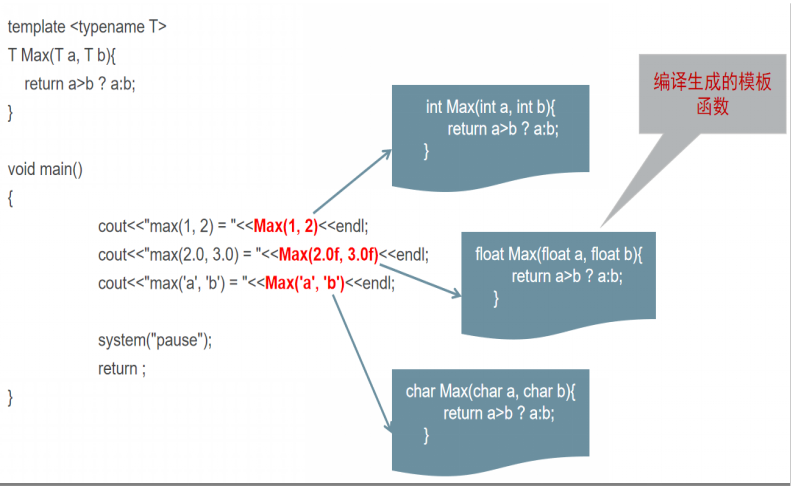也就是，在我们编译的时候，编译器内部会自动生成这些模板函数，然后再进行调用，当然，这些都是编译器内部生成的，我们可以不用去了解太深，我们知道这个概念就可以了。

如果我们来比较类的两个对象

#include <iostream>

using namespace std;

//定义一个类
class Son {
public:
Son(int age) { m_age = age; }
~Son(){}

int getAge() { return m_age; }

private:
int m_age;
};

//template 关键字告诉C++编译器 要开始泛型编程了
//T - 参数化数据类型
template <typename T, typename T2>
T Max(T a, T2 b) {
return a > b ? a : b;
}

int main(void){
Son s1(18);
Son s2(21);

cout << "Max(s1, s2): " << Max(s1, s2).getAge() << endl;
/*
* 模板函数 在类的对象中， 不认识 > 这个符号
Son Max(Son s1, Son s2){
return a > b ? a : b;
}
*/
return 0;
}


就会出现这样的错误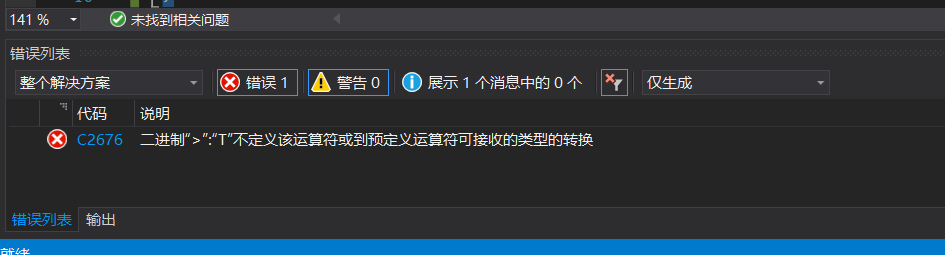因为在类对象的比较中，不认识这个 > 的符号，所以我们想要能成功执行，我们得在类中实现 > 的运算符重载

#include <iostream>

using namespace std;

//定义一个类
class Son {
public:
Son(int age) { m_age = age; }
~Son(){}

int getAge() { return m_age; }

//重载 > 运算符
bool operator >(Son& res) {
if (this->m_age > res.m_age) return true;

return false;
}

private:
int m_age;
};

//template 关键字告诉C++编译器 要开始泛型编程了
//T - 参数化数据类型
template <typename T, typename T2>
T Max(T a, T2 b) {
return a > b ? a : b;
}

int main(void){
Son s1(18);
Son s2(21);

cout << "Max(s1, s2): " << Max(s1, s2).getAge() << endl;
/*
* 模板函数 在类的对象中， 不认识 > 这个符号
Son Max(Son s1, Son s2){
return a > b ? a : b;
}
*/
return 0;
}


这样就可以执行得出结果了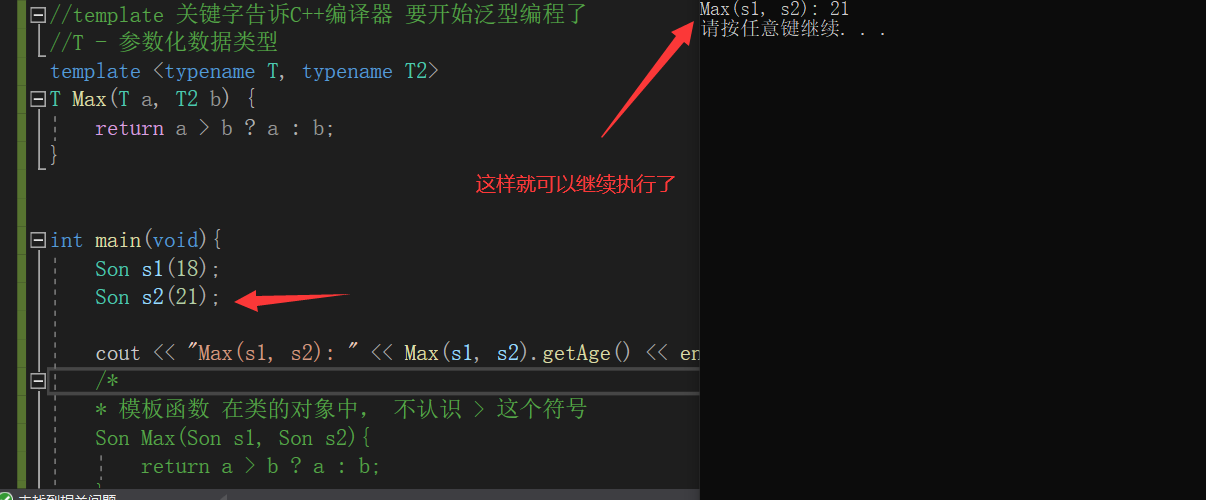# 函数模板和函数重载

我们先来看一下这个demo

#include <iostream>

using namespace std;

template <typename T>
void Test(T& a, T& b) {
T c;
c = a;
a = b;
b = c;

cout << "Test 函数模板被调用了.." << endl;
}

void Test(int& a, int& b) {
int c;
c = a;
a = b;
b = c;

cout << "Test 普通函数被调用.." << endl;
}

int main(void) {
int n = 99;
int m = 65;

Test(n, m);

return 0;
}


这是来观察，当存在函数模板和普通对应的函数的时候，会调用哪个函数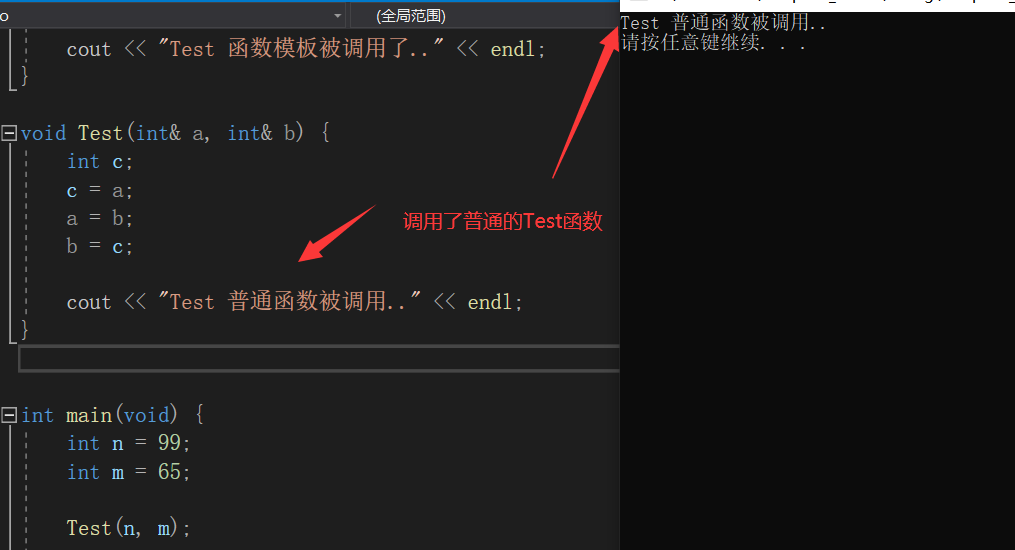可以看到 当函数模板和普通函数并存的时候，参数类型会和普通重载函数更加匹配

我们把普通函数注释掉，我们才能看到调用函数模板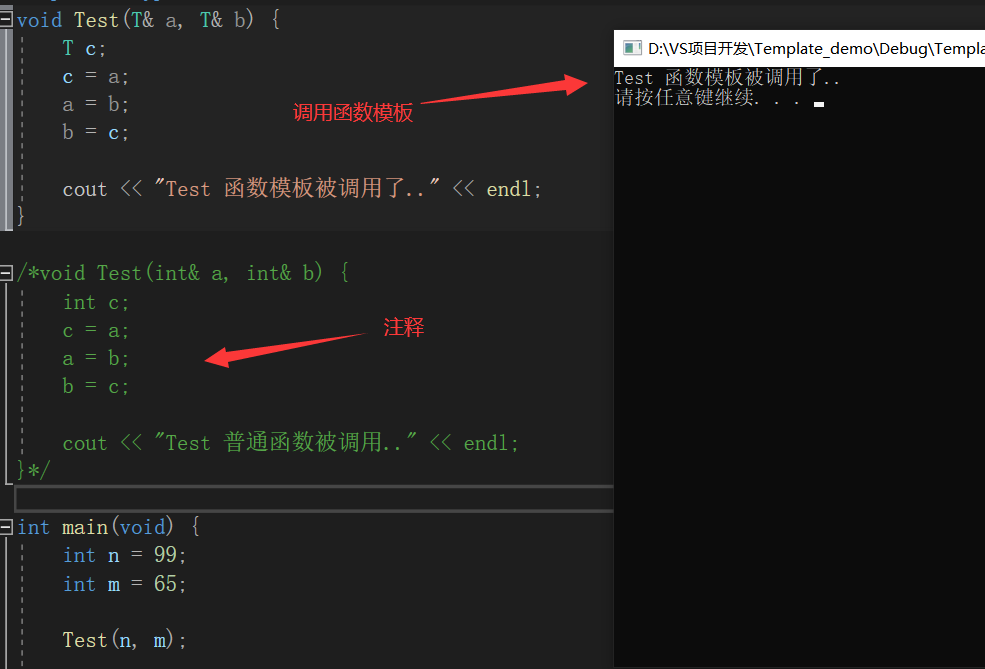如果普通函数和函数模板都在的情况下，我们非要使用函数模板，这个怎么实现呢，很简单，如果显式的使用函数模板,则使用<> 类型列表。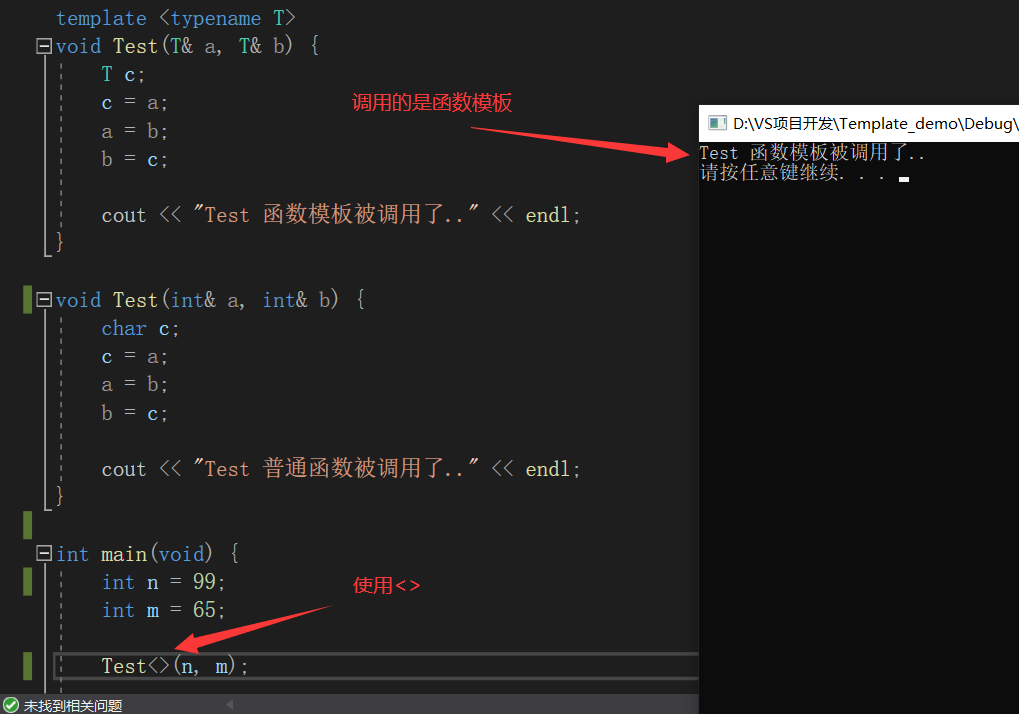如果我们将一个传递的参数改为别的类型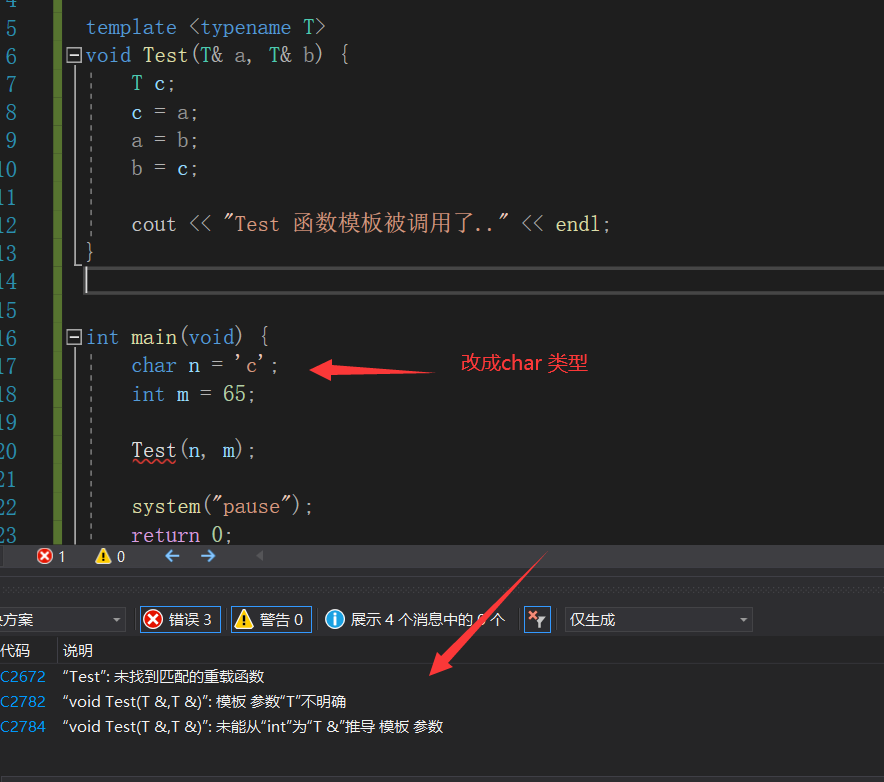不存在对应的普通函数，函数模板不会提供隐式的类型转换，必须是严格的类型匹配

函数模板和普通函数区别结论：

两者允许并存
函数模板不允许自动类型转化

如果有函数模板和普通函数的时候，普通函数的参数类型不不一致的时候，也会调用普通函数，因为会有隐式的转换，但是如果有函数模板的时候，函数模板会产生更好的匹配，使用函数模板。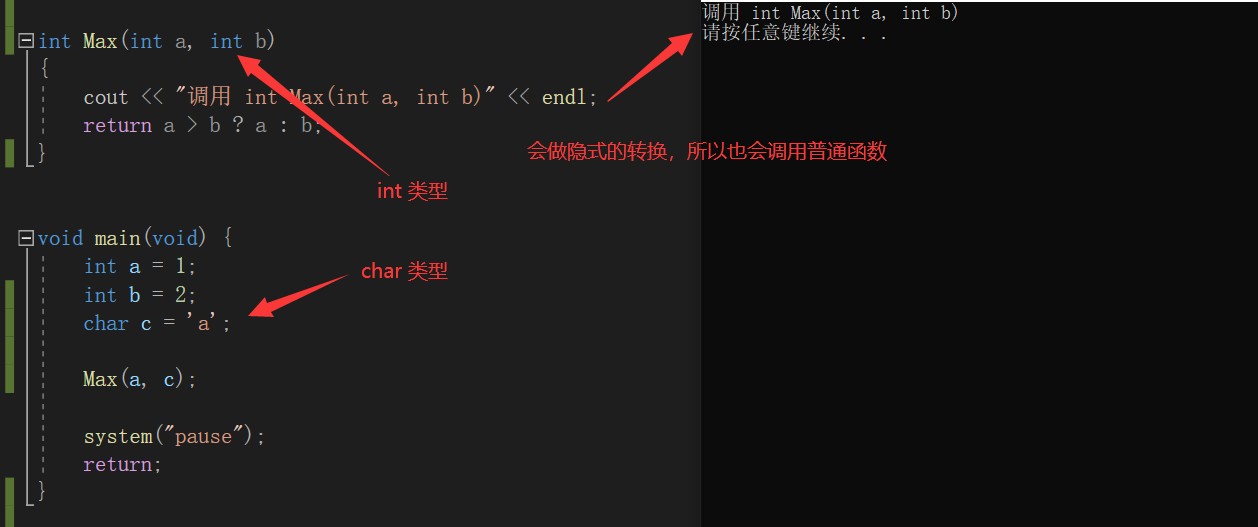有更好的函数模板的时候，会选择函数模板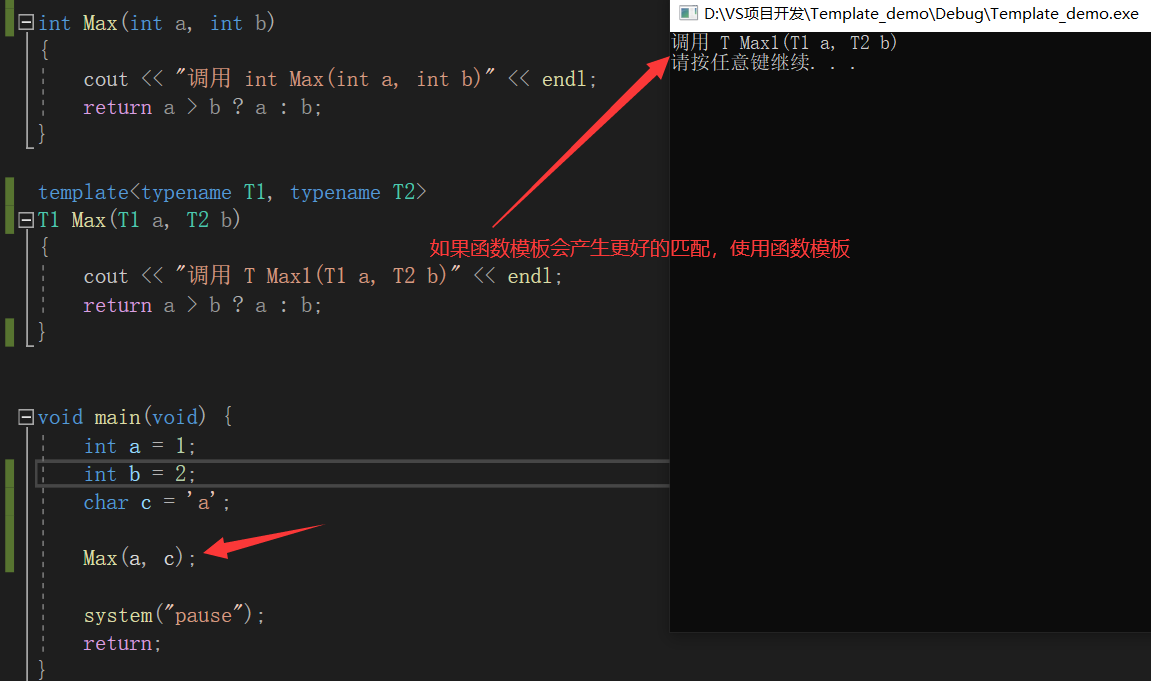## 嵌套使用函数模板

在函数模板中，我们也可以嵌套使用函数模板。

#include <iostream>

using namespace std;

template<typename T>
T Max(T a, T b)
{
cout << "调用 T Max(T a, T b)" << endl;
return a > b ? a : b;
}

template <typename T>
T Max(T a, T b, T c) {
cout << "调用 T Max(T a, T b, T c)" << endl;
return Max(Max(a, b), c);
}

int main(void) {
int a = 1;
int b = 2;
int c = 3;

Max(a, b, c);

system("pause");
return 0;
}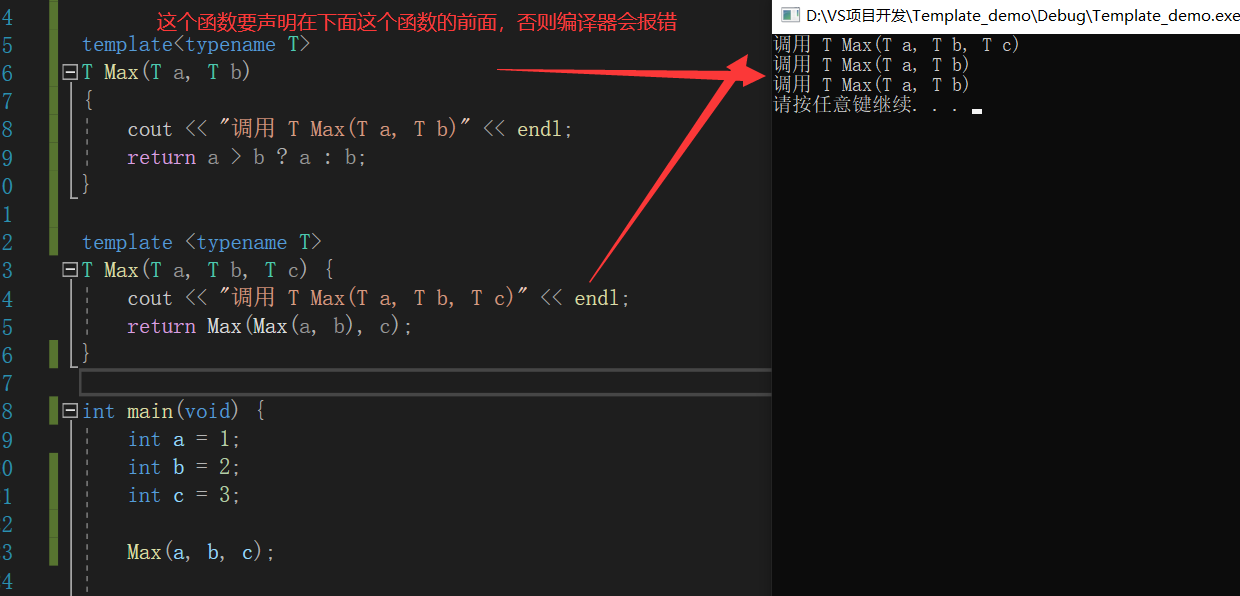注意：嵌套中的函数要声明在这个函数之前，否则编译器会找不到哪个函数，然后会报错

# 函数模板和普通函数在一起，调用规则

1 函数模板可以像普通函数一样被重载
2 C++编译器优先考虑普通函数
3 如果函数模板可以产生一个更好的匹配，那么选择模板
4 可以通过空模板实参列表的语法限定编译器只通过模板匹配

# 在Linux中反汇编查看函数模板被调用的机制

在Linux创建一个cpp文件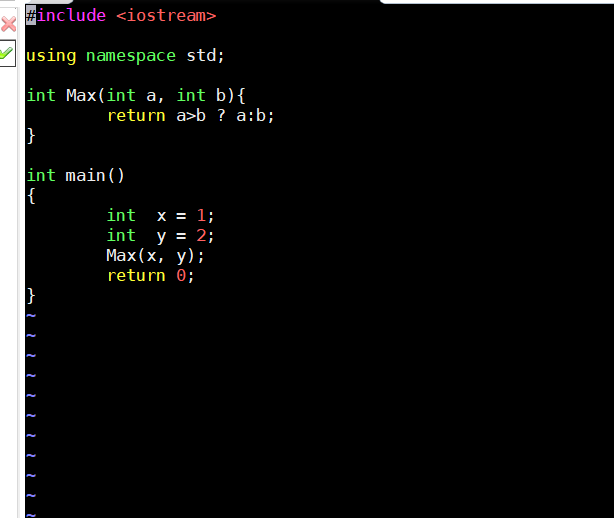保存退出之后编译生成指定的汇编的文件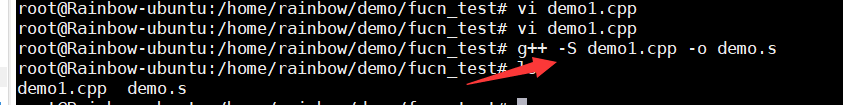然后查看这个汇编文件，找到我们熟悉的main函数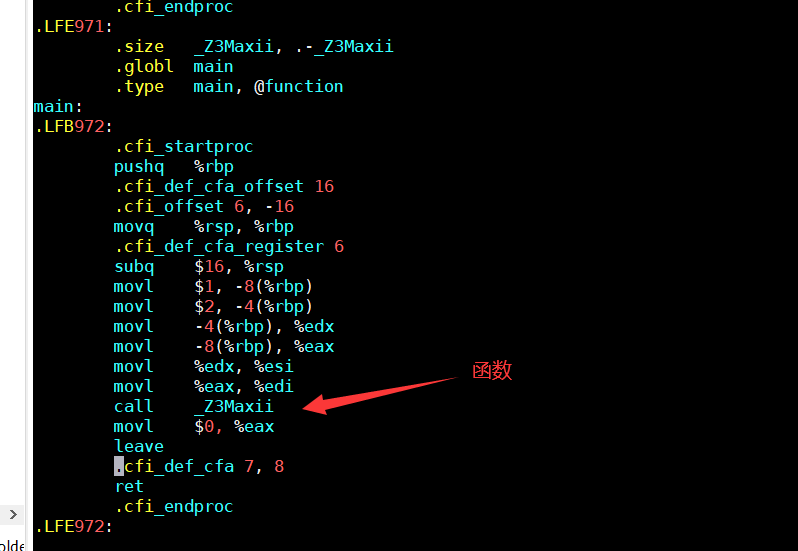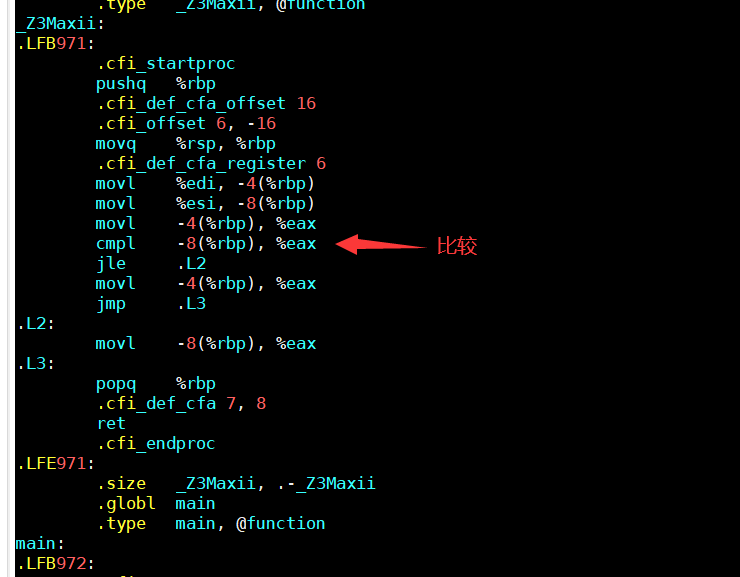再执行一个有函数模板的CPP文件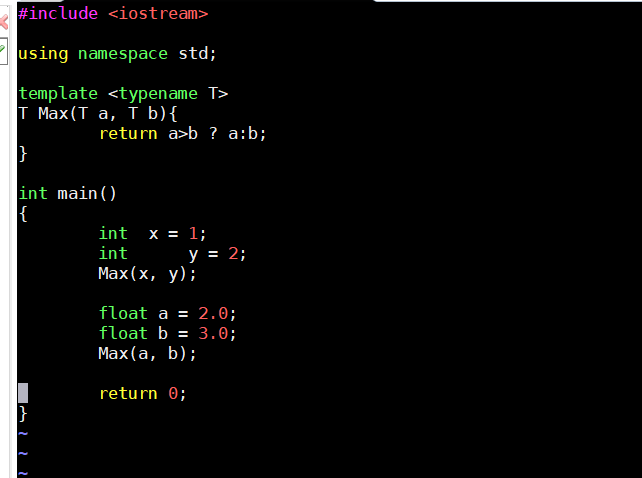在main函数中调用了函数模板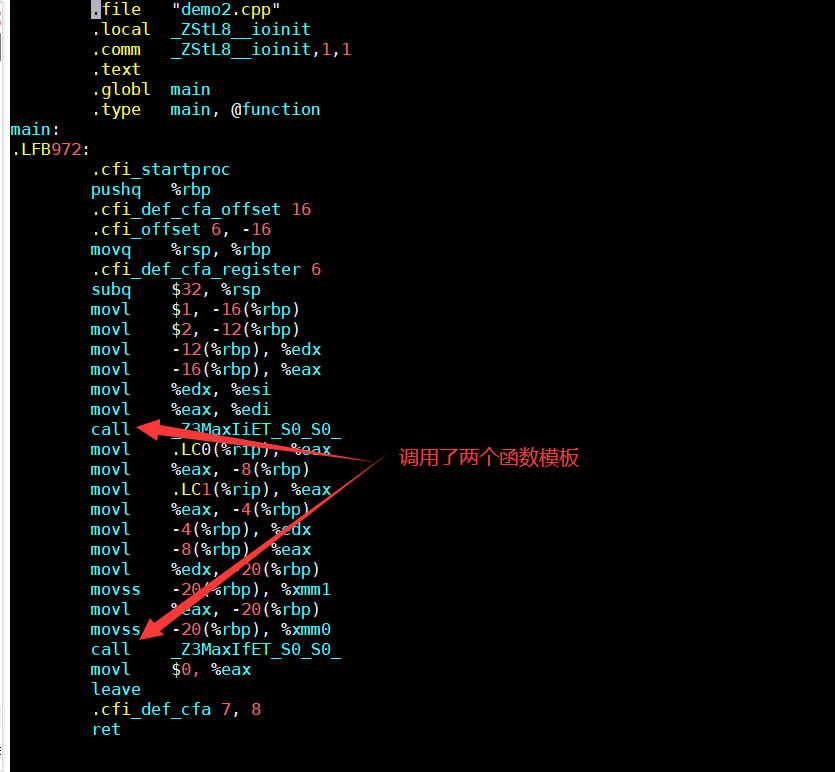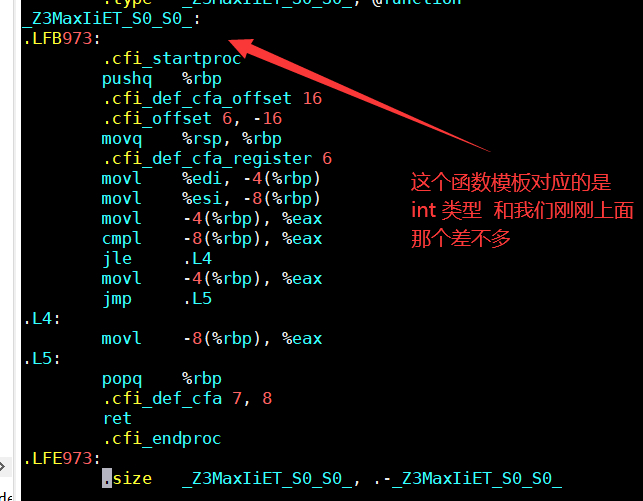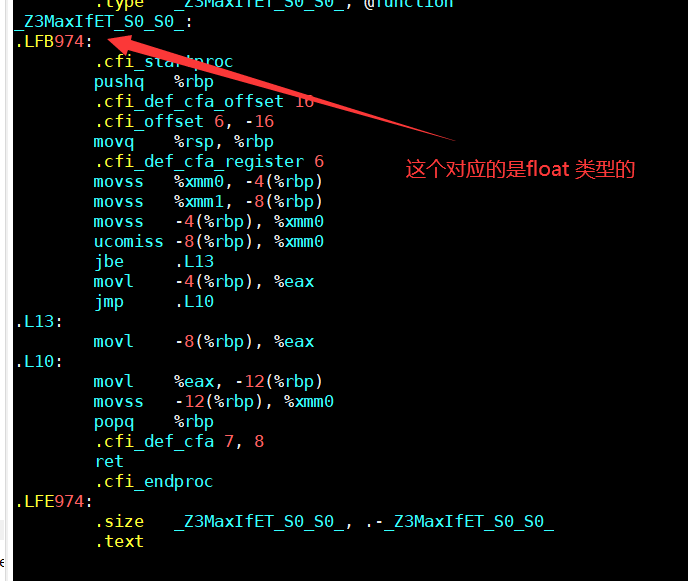从上面可以看出，我们只是定义了一个函数模板，而在调用的时候，内部会自动帮我们生成一个模板函数，然后再执行这个模板函数，得到我们想要的结果

结论

1. 编译器并不是把函数模板处理成能够处理任意类型的函数
2. 编译器从函数模板通过具体类型产生不同的函数
展开全文c++ linux
• *1. 运用函数重载机制定义三个函数，函数名为Substract每个函数的参数分别有...2.设计一个函数模板，函数名sub用于完成两个数相减，并测试三种不同类型的模板函数。请体会1和2的区别。（函数重载和函数模板各自的特点）
•  像上面的这个例子，模板函数的声明在头文件内，用到这个模板函数的另一个源文件A中包含了这个头文件（但是没有包含模板函数的定义所在的文件）时，编译器只看到了声明，这时候并没有把函数的定义实例化出来，...

参考：

https://www.cnblogs.com/cnsec/p/3789824.html

## general的编译链接过程：

举个例子：

//---------------test.h-------------------//
void f();//这里声明一个函数f

//---------------test.cpp--------------//
＃include”test.h”
void f()
{
…//do something
} //这里实现出test.h中声明的f函数

//---------------main.cpp--------------//
＃include”test.h”
int main()
{
f(); //调用f，f具有外部连接类型
} 

在这个例子中，test. cpp和main.cpp各被编译成为不同的.obj文件[姑且命名为test.obj和main.obj]，在main.cpp中，调用了f函数，然而当编译器编译main.cpp时，它所仅仅知道的只是main.cpp中所包含的test.h文件中的一个关于void f();的声明，所以，编译器将这里的f看作外部连接类型，即认为它的函数实现代码在另一个.obj文件中，本例也就是test.obj，也就是说，main.obj中实际没有关于f函数的哪怕一行二进制代码，而这些代码实际存在于test.cpp所编译成的test.obj中。在main.obj中对f的调用只会生成一行call指令，像这样： call f （C++中这个名字当然是经过处理的）

在编译时，这个call指令显然是错误的，因为main.obj中并无一行f的实现代码。那怎么办呢？这就是连接器的任务，连接器负责在其它的.obj中[本例为test.obj]寻找f的实现代码，找到以后将call f这个指令的调用地址换成实际的f的函数进入点地址。需要注意的是：连接器实际上将工程里的.obj“连接”成了一个.exe文件，而它最关键的任务就是上面说的，寻找一个外部连接符号在另一个.obj中的地址，然后替换原来的“虚假”地址。

这个过程如果说的更深入就是： call f这行指令其实并不是这样的，它实际上是所谓的stub，也就是一个jmp 0x23423[这个地址可能是任意的，然而关键是这个地址上有一行指令来进行真正的call f动作。也就是说，这个.obj文件里面所有对f的调用都jmp向同一个地址，在后者那儿才真正”call”f。这样做的好处就是连接器修改地址时只要对后者的call XXX地址作改动就行了。但是，连接器是如何找到f的实际地址的呢[在本例中这处于test.obj中]，因为.obj于.exe的格式都是一样的，在这样的文件中有一个符号导入表和符号导出表[import table和export table]其中将所有符号和它们的地址关联起来。这样连接器只要在test.obj的符号导出表中寻找符号f[当然C++对f作了mangling]的地址就行了，然后作一些偏移量处理后[因为是将两个.obj文件合并，当然地址会有一定的偏移，这个连接器清楚]写入main.obj中的符号导入表中f所占有的那一项。

这就是大概的过程。其中关键就是： 编译main.cpp时，编译器不知道f的实现，所有当碰到对它的调用时只是给出一个指示，指示连接器应该为它寻找f的实现体。这也就是说main.obj中没有关于f的任何一行二进制代码。 编译test.cpp时，编译器找到了f的实现。于是乎f的实现[二进制代码]出现在test.obj里。 连接时，连接器在test.obj中找到f的实现代码[二进制]的地址[通过符号导出表]。然后将main.obj中悬而未决的call XXX地址改成f实际的地址。

完成。

## 模板的编译链接过程：

然而，模板函数的代码其实并不能直接编译成二进制代码，其中要有一个“具现化”的过程。举个例子：

//----------main.cpp------//
template<class T>
void f(T t) {}

int main()
{
…//do something
f(10); //call f<int> 编译器在这里决定给f一个f<int>的具现体
…//do other thing
} 

也就是说，如果你在main.cpp文件中没有调用过f，f也就得不到具现，从而main.obj中也就没有关于f的任意一行二进制代码！！如果你这样调用了：

f(10); //f<int>得以具现化出来

f(10.0); //f<double>得以具现化出来

这样main.obj中也就有了f<int>,f<double>两个函数的二进制代码段。以此类推。

然而具现化要求编译器知道模板的定义，不是吗？

看下面的例子：[将模板和它的实现分离]

//-------------test.h----------------//
template<class T>
class A
{
public:
void f(); //这里只是个声明
};

//---------------test.cpp-------------//
＃include”test.h”
template<class T>
void A<T>::f() //模板的实现，但注意：不是具现
{
…//do something
}

//---------------main.cpp---------------//
＃include”test.h”
int main()
{
A<int> a;
a. f(); //编译器在这里并不知道A<int>::f的定义，因为它不在test.h里面
//于是编译器只好寄希望于连接器，希望它能够在其他.obj里面找到
//A<int>::f的实现体,在本例中就是test.obj，然而，后者中真有A<int>::f的
//二进制代码吗？NO！！！因为C++标准明确表示，当一个模板不被用到的时
//侯它就不该被具现出来，test.cpp中用到了A<int>::f了吗？没有！！所以实
//际上test.cpp编译出来的test.obj文件中关于A::f的一行二进制代码也没有
//于是连接器就傻眼了，只好给出一个连接错误
//但是，如果在test.cpp中写一个函数，其中调用A<int>::f，则编译器会将其
//具现出来，因为在这个点上[test.cpp中]，编译器知道模板的定义，所以能够具现化，
//于是，test.obj的符号导出表中就有了A<int>::f这个符号的地址，于是连接器就能
//够完成任务。

}

关键是：在分离式编译的环境下，编译器编译某一个.cpp文件时并不知道另一个.cpp文件的存在，也不会去查找[当遇到未决符号时它会寄希望于连接器]。这种模式在没有模板的情况下运行良好，但遇到模板时就傻眼了，因为模板仅在需要的时候才会具现化出来，所以，当编译器只看到模板的声明时，它不能具现化该模板，只能创建一个具有外部连接的符号并期待连接器能够将符号的地址决议出来。然而当实现该模板的.cpp文件中没有用到模板的具现体时，编译器懒得去具现，所以，整个工程的.obj中就找不到一行模板具现体的二进制代码，于是连接器也黔驴技穷了。

总结：

像上面的这个例子，模板函数的声明在头文件内，用到这个模板函数的另一个源文件A中包含了这个头文件（但是没有包含模板函数的定义所在的文件）时，编译器只看到了声明，这时候并没有把函数的定义实例化出来，编译器直接把找到函数定义的工作甩锅给链接器。但是链接器拿到的所有.obj文件中都没有这个模板函数的实例化定义，所以链接的时候就找不到。

如果模板函数的定义也在头文件内，编译器在编译的时候就直接拿着模板实例化出这个模板函数的定义，并把这份定义存放在编译输出的.obj文件内。

所以其实模板的实例化都是在编译期完成的，如果在编译的时候，找不到模板函数的定义，就先不在这一次编译中实例化该模板函数，直接生成一个不含这个模板函数实例化结果的.obj文件，等到与其他.obj文件链接的时候再链接到一起。

因此只要让编译器能够在某一次编译时看到模板函数的定义并将其实例化出来，最后把这些编译得到的目标文件链接在一起，就不会有模板函数链接失败的问题。例如可以：（1）将模板函数的定义直接写在头文件内；（2）可以写在源文件B内，并将这个源文件B包含在源文件A内；（3）在源文件A内想办法触发这个模板函数的实例化（例如可以显式实例化：template void f<int>();）在编译A时将实例化结果写入生成的.obj文件内，让B的.obj文件与A的.obj文件链接时能够找到实例化结果。

上面的三种方法，前两种都是在编译期让所有用到模板函数的地方直接实例化函数定义，这样做会使每一个编译结果都包含实例化结果，导致目标文件较大，链接的时候需要把重复的实例化定义去重，编译链接的时间也会长一些。使用第三种方法，可以用一个特定的.cc文件显示实例化所有会被用到的模板实例，单独编译这个文件，最后让它参与链接，用这种方法，不会产生巨大的头文件，加快编译速度。而且头文件本身也显得更加“干净”和更具有可读性。但这个方法不能得到惰性实例化的好处，即它将显式地生成所有的成员函数，另外还要维护一个这样的文件。所以为了容易使用，几乎总是在头文件中放置全部的模板声明和定义。

展开全文C++ 编译
• 函数模板：函数的重载能够实现一个函数名多用，将实现相同或者相似功能的函数用同一个函数名来...模板函数：为函数模板传参，根据实际传入的参数类型生成的一个重载函数即为模板函数，它是函数模板的一次实例化。 ...c++
• 模板类与类模板、函数模板与模板函数等的区别 函数指针 = 指向函数的指针 指针函数=返回指针的函数 数组指针=指向数组的指针 指针数组=内容是指针的数组 类模板=用来产生类的模板 模板类=使用类模板产生的类...
• 今天在写代码时，遇到了模板和特化，在网上找了资料后问题呗一一解决，转载...特化函数与模板函数的区别： (1)、模板函数的T参数只能传入类类型的参数；特化函数的参数只能传入对应的参数类型，基本类型或类类型。...
• 函数模板与模板函数 函数模板是一组函数的抽象描述，它不是一个实实在在的函数，函数模板不会编译成任何目标代码。函数模板必须先实例化成模板函数，这些模板函数再程序运行时会进行编译和链接，然后产生相应的...
• 1.函数模板的声明和模板函数的生成 1.1函数模板的声明 函数模板可以用来创建一个通用的函数，以支持多种不同的形参，避免重载函数的函数体重复设计。它的最大特点是把函数使用的数据类型作为参数。 函数模板的...
• ## C++模板函数 & 模板类

千次阅读 多人点赞 2017-12-18 16:36:32C++
• 1.分离编译模式 ...下面的程序由三个文件组成：func.h用来对函数模板进行申明，func.cpp用来定义函数模板，main.cpp包含func.h头文件并调用相应的函数模板。 /***func.h***/ template<class> void
• C++类中的虚函数不能为模板函数 首先，理解模板的原理。例如，定义了一个模板函数： template<typename A> void Func(const A& a) { std::cout <<"Func(const A& a) : "<< a <<...c++ 开发语言 后端
• ## 关于C++模板函数声明与定义的问题

万次阅读 多人点赞 2018-07-22 21:59:37
关于C++模板函数声明与定义的问题 关于C++模板函数声明与定义的问题 模板函数出现的问题 模板函数问题解决 模板函数出现的问题 今天在写代码的时候，发现了一个关于模板函数的问题。如下所示， demo...C++
• 主要介绍了golang模板template自定义函数用法,结合实例形式分析了Go语言模板自定义函数的基本定义与使用方法,需要的朋友可以参考下
• 最近要写一个模板函数，要求是必须被指定的类型才能执行，未被指定的类型要求报错。 注册器模板类 其实所谓接受指定类型作为参数就是对类型进行注册，凡是注册过的类型才能传入模板，非注册类型应当匹配失败，所以...
• 1.函数qSwap()用来交换两个变量的值，例如： QString second( "Einstein" ); QString name( "Albert" ); qSwap( second, name ); 函数qCount()用于统计容器中一个值出现的次数。例如 QValueList<int> l; ...
• 模板函数是使用于模板文件的处理函数，模板函数的使用方式是{% 模板函数 %} 1. 创建自定义函数文件夹 想要使用自定义模板函数的话需要先创建用于存放函数的文件夹，而在django中对于自定义函数文件夹的名称有严格的...
• C++函数模板 我们知道，数据或数值可以通过函数参数传递，在函数定义时它们是未知的，只有在发生函数调用时才能确定其值。这就是数据的参数化。 其实，数据类型也可以通过参数来传递，在函数定义是可以不指明具体的...
• 相信很多刚入门c++的同学在学数据结构或者查看大佬的代码中，我们都会看到: template <typename T> 类模板; ...函数模板： ...本文只是简单说明一下我们使用类模板函数模板需要注意什么（因为我...
• c++模板不支持分离编译, 把你模板类的声明和实现放到.h文件里面 。按照这个说的把.h和.cpp文件合并后，果然可以了。 但是为什么呢，为什么模板就不支持分离编译？---继续google ing 搜到了如下文章（文章原文链接...
• 一、类模板全特化、偏特化 #pragma once #include #include template class TC { public: TC() { std::cout << "泛化版本构造函数" < class TC { public: TC() { std::cout << "全特化版本...
• 1，一个普通类的一个成员函数可以成为模板成员函数么？ 答案是可以的，实例如下 #include<iostream> #include<string> using namespace std; class PrintIt { public: PrintIt(ostream &os) :_os...python c++ java 算法
• ## 模板函数调用

千次阅读 2018-04-24 17:18:02
#include&lt;iostream&gt;using namespace std;template &lt;class t&gt;t MAX(t a,t b){ cout&lt;&lt;"模板一被调用"&lt;&lt;endl; }template&...函数
• 很少遇到bind类成员模板函数的，我也是在阅读buttonrpc_cpp14源码时遇到的相关用法。不得不佩服buttonrpc_cpp14的作者，把c++11和C++14的新功能玩得真叫一个6。 话不多说直接上代码： class ThreadObject { ...
• 后置返回值类型主要用于模板函数中，它是C++11推出的新用法。其中使用到了auto和decltype两种类型说明符。 auto和decltype虽然都是类型说明符，但是二者是不同的：auto是根据推导初始值的类型来确定变量的类型，而...
• 比如我们现在有一个需求，要实现两个数字交换，这两个数字可能是整型，浮点型或者其他类型，这时我们可能会考虑编写一个函数实现其交换的功能，但是由于数据类型不同，我们可能会将copy一遍，仅仅是替换不同的类型。...宏定义函数...